## 1 Introduction

Research on regularity of weak solutions or minimizers of the problems

\begin{aligned} \mathrm {div}A(x, Du)=0 \quad \text {and}\quad \min _u \int _\Omega F(x,Du)\,\mathrm{d}x \end{aligned}

is a major topic in the partial differential equations and the calculus of variations. If there is no direct dependence on x (i.e., $$A(x,\xi )\equiv A(\xi )$$ and $$F(x,\xi )\equiv F(\xi )$$), these are called autonomous problems. The simplest non-linear model cases is the p-power function $$F(\xi )=|\xi |^p$$, $$1<p<\infty$$, and the corresponding Euler–Lagrange equation is the p-Laplace equation where $$A(Du)=|Du|^{p-2}Du$$. The maximal regularity of weak solutions of the p-Laplace equation is $$C^{1,\alpha }$$ for some $$\alpha \in (0,1)$$ depending only on p and the dimension n (e.g., [14, 17, 31, 39]).

For non-autonomous problems, there is also direct x-dependence. To tackle this case, Giaquinta and Giusti [20, 21] introduced the following p-type growth conditions:

\begin{aligned} {\left\{ \begin{array}{ll} \xi \mapsto F(x,\xi )\text { is } C^2, \\ \nu |\xi |^p \leqslant F(x,\xi )\leqslant L(1+|\xi |^p), \\ \nu (\mu ^2+|\xi |^2)^{\frac{p-2}{2}}|\lambda |^2 \leqslant D_\xi ^2F(x,\xi )\lambda \cdot \lambda \leqslant L (\mu ^2+|\xi |^2)^{\frac{p-2}{2}} |\lambda |^2, \\ |F(x,\xi )-F(y,\xi )| \leqslant \omega (|x-y|)(1+|\xi |^p). \end{array}\right. } \end{aligned}
(1.1)

Here, F is related to the perturbed case $$a(x)|\xi |^p$$ and has the same p-type growth at all points. Lieberman  generalized the model by replacing $$|\xi |^p$$ with Orlicz growth $$\varphi (|\xi |)$$. Marcellini  introduced non-standard, so-called (pq)-growth where the exponent p on the right-hand side is replaced by $$q>p$$. In this situation, we need to assume that $$\frac{q}{p}$$ is close to 1, see, e.g., [3, 4, 12, 35]. However, all these structure conditions fail to accommodate many kinds of energy functionals since the variability in the x- and $$\xi$$-direction are treated separately.

For many years, it was thought that the only way to treat the x- and $$\xi$$-directions together was through special cases. Consequently, a plethora of studies deal with the variable exponent case $$F(x,\xi )=|\xi |^{p(x)}$$. Over the past half-dozen years the double phase functional $$F(x,\xi )=|\xi |^p + a(x) |\xi |^q$$, $$1<p\leqslant q$$ and $$a\geqslant 0$$, has attracted much attention. These models were first studied by Zhikov [40, 41] in the 1980’s in relation to Lavrentiev’s phenomenon and have been considered in thousands of papers [36, 38]. Moreover, various variants and borderline cases have been investigated, such as: perturbed variable exponent $$|\xi |^{p(x)} \log (e+|\xi |)$$; Orlicz variable exponent $$\psi (|\xi |)^{p(x)}$$ or $$\psi (|\xi |^{p(x)})$$; degenerate double phase $$|\xi |^p + a(x) |\xi |^p \log (e+|\xi |)$$; Orlicz double phase $$\varphi (|\xi |) + a(x) \psi (|\xi |)$$; triple phase $$|\xi |^p + a(x) |\xi |^q + b(x) |\xi |^r$$; double variable exponent $$|\xi |^{p(x)}+|\xi |^{q(x)}$$; and variable exponent double phase $$|\xi |^{p(x)} + a(x) |\xi |^{q(x)}$$; see  for references. We emphasize that all these special cases are covered by our results.

In , we introduced a different approach which does not impose any direct restriction on $$\frac{q}{p}$$. However, we were only able to prove maximal regularity for local minimizers when $$F(x,\xi )=F(x,|\xi |)$$ has so-called Uhlenbeck structure. In this article we extend the results to both minimizers and weak solutions and dispense with the Uhlenbeck restriction.

We collect some conditions for $$A:\Omega \times \mathbb {R}^n\rightarrow \mathbb {R}^n$$ and $$F:\Omega \times \mathbb {R}^n\rightarrow \mathbb {R}$$ with an open set $$\Omega \subset \mathbb {R}^n$$ ($$n\geqslant 2$$), which determine our equation and minimization energy, respectively; see Sect. 2 for further definitions and notation, including the continuity assumption (wVA1), with is the other main assumption.

### Assumption 1.1

We say that $$A:\Omega \times \mathbb {R}^n\rightarrow \mathbb {R}^n$$ satisfies Assumption 1.1 if the following three conditions hold:

1. (1)

For every $$x\in \Omega$$, $$A(x, 0)= 0$$, $$A(x,\cdot )\in C^{1}(\mathbb {R}^n\setminus \{ 0\}, \mathbb {R}^n)$$ and for every $$\xi \in \mathbb {R}^n$$, $$A(\cdot ,\xi )$$ is measurable.

2. (2)

There exist $$L\geqslant 1$$ and $$1<p<q$$ such that the radial function $$t\mapsto | D_\xi A(x,te)|$$ satisfies (A0), (aInc)$$_{p-2}$$ and (aDec)$$_{q-2}$$ with the constant L, for every $$x\in \Omega$$ and $$e\in {\mathbb {R}^n}$$ with $$|e|=1$$. (The (pq)-growth condition)

3. (3)

There exists $$L\geqslant 1$$ such that

\begin{aligned} | D_\xi A(x,\xi ')| \leqslant L\, D_\xi A(x,\xi )e \cdot e \end{aligned}

for all $$x\in \Omega$$ and $$\xi ,\xi ',e\in {\mathbb {R}^n}$$ with $$|\xi |=|\xi '|\ne 0$$ and $$|e|=1$$. (The quasi-isotropic ellipticity condition)

The (A0) condition in (2) means that a coefficient factor of A is nondegenerate and nonsingular, for instance $$a\approx 1$$ when $$A(x,\xi )=a(x) |\xi |^{p-2}\xi$$. The (aDec)$$_{q-2}$$ and (aInc)$$_{p-2}$$ conditions in (2) are equivalent to the function $$t\mapsto t^2| D_\xi A(x,te)|$$ satisfying the $$\Delta _2$$- and $$\nabla _2$$-conditions, respectively. In particular, we note from (2) that $$D_\xi A(x,\xi )\ne 0$$ when $$\xi \ne 0$$. Uhlenbeck structure has been replaced by (3), which is a quasi-isotropy condition since different directions behave the same up to a constant. It is known that completely anisotropic equations do not necessarily have any regularity as solutions may even be locally unbounded . We also note that if $$A(x,\xi )=D_\xi F(x,\xi )$$ the condition (3) means that the Hessian matrix $$D_\xi ^2 F(x,\xi )$$ with $$\xi \ne 0$$ is positive definite and all its eigenvalues on each sphere for $$\xi$$ are comparable uniformly in x and the radii of spheres, that is,

\begin{aligned} 1\leqslant \frac{\sup \{\text {eigenvalues of }D_\xi ^2 F(x,te) : |e|=1\}}{\inf \{\text {eigenvalues of }D_\xi ^2 F(x,te) : |e|=1\}} \leqslant \tilde{L} \quad \text {for each }\ x\in \Omega \ \text { and }\ t>0, \end{aligned}

where $$\tilde{L}$$ depends only on L and n. Compare this to the p-growth condition in (1.1), which implies a stronger condition, where x is inside the supremum and infimum:

\begin{aligned} 1\leqslant \frac{\sup \{\text {eigenvalues of }D_\xi ^2 F(x,te) : x\in \Omega ,\, |e|=1\}}{\inf \{\text {eigenvalues of }D_\xi ^2 F(x,te) : x\in \Omega ,\ |e|=1\}} \leqslant \tilde{L} \quad \text {for each }\ t>0. \end{aligned}

We further refer to [12, 13] for a discussion of non-uniformly elliptic conditions in terms of ratios of eigenvalues (in particular [12, Sect. 4.6] and [13, Sect. 1]) and related regularity results (see also Remark 1.7).

With these assumptions we consider the following quasilinear elliptic equation in divergence form:

We say that $$u\in W^{1,1}_{\mathrm {loc}}(\Omega )$$ is a local weak solution if $$|Du|\,|A(\cdot ,Du)|\in L^1_{\mathrm {loc}}(\Omega )$$ and

\begin{aligned} \int _{\Omega } A(x,Du)\cdot D\zeta \,\mathrm{d}x = 0\quad \text {for all }\ \zeta \in C^\infty _0(\Omega ). \end{aligned}

We show that such solutions are quasiminimizers of a related functional with Uhlenbeck structure; thus $$C^{0,\alpha }$$-regularity for some $$\alpha \in (0,1)$$ and higher integrability follow from the results in [24, 26], see Theorem 4.1. Let us state the first main maximal regularity theorem for general weak solutions.

### Theorem 1.2

(Maximal regularity for solutions) Let $$A:\Omega \times {\mathbb {R}^n} \rightarrow {\mathbb {R}^n}$$ satisfy Assumption 1.1 and let $$u\in W^{1,1}_{\mathrm {loc}}(\Omega )$$ be a local weak solution to (div A). Define $$A^{(-1)}(x,\xi ){:=}|\xi |A(x,\xi )$$.

1. (1)

If $$A^{(-1)}$$ satisfies (wVA1), then $$u\in C^{0,\alpha }_{\mathrm {loc}}(\Omega )$$ for every $$\alpha \in (0,1)$$.

2. (2)

If $$A^{(-1)}$$ satisfies (wVA1) with $$\omega _\varepsilon (r)\leqslant r^{\beta _\varepsilon }$$ for some $$\beta _\varepsilon >0$$, then $$u\in C^{1,\alpha }_{\mathrm {loc}}(\Omega )$$ for some $$\alpha =\alpha (n,p,q,L, \bar{L},\beta _\varepsilon )\in (0,1)$$.

### Remark 1.3

The continuity condition (wVA1) for $$\Phi$$-functions was introduced in , see also Sect. 2.2. In our knowledge, the above theorem covers all previous known $$C^{1,\alpha }$$-regularity results for equation ($$\mathrm {div}A$$) with $$\alpha$$ independent of the solution. Some examples of functionals satisfying Assumptions 1.1 or 1.5 are presented in Sect. 7.

### Remark 1.4

In (wVA1), $$\varepsilon >0$$ is arbitrary and it is possible that $$\beta _\varepsilon \rightarrow 0$$ as $$\varepsilon \rightarrow 0$$. Moreover, the constant $$\bar{L}=\bar{L}_K$$ is from (wVA1) and $$K>0$$ is arbitrary. However, for given structure we actually choose certain, positive $$\varepsilon$$ and K (see Sect. 6, in particular, (6.1) and (6.2)), and $$\alpha$$ in the previous theorem depends on this $$\beta _\varepsilon$$ and $$\bar{L}=\bar{L}_K$$. The same applies in Theorem 1.6.

If equation ($$\mathrm {div}A$$) is an Euler–Lagrange equation, that is, if $$A=D_\xi F$$ for some a function $$F:\Omega \times \mathbb {R}^n\rightarrow \mathbb {R}$$, then we can consider milder regularity assumptions in the context of variational calculus.

### Assumption 1.5

We say that $$F:\Omega \times \mathbb {R}^n\rightarrow [0,\infty )$$ satisfies Assumption 1.5 if the following two conditions hold:

1. (1)

For every $$x\in \Omega$$, $$F(x, 0)=|D_\xi F(x,0)|= 0$$, $$F(x,\cdot )\in C^{1}(\mathbb {R}^n)\cap C^{2}(\mathbb {R}^n\setminus \{ 0\})$$ and for every $$\xi \in \mathbb {R}^n$$, $$F(\cdot ,\xi )$$ is measurable.

2. (2)

The derivative $$A{:=}D_\xi F$$ satisfies conditions (2) and (3) of Assumption 1.1.

From this assumption it follows that $$F(x,\xi )>0$$ for all $$x\in \Omega$$ and $$\xi \in {\mathbb {R}^n} \setminus \{0\}$$, see (3.7) below. We say that $$u\in W^{1,1}_{\mathrm {loc}}(\Omega )$$ is a local minimizer if $$F(\cdot ,Du)\in L^1_{\mathrm {loc}}(\Omega )$$ and

for every $$v\in W^{1,1}_{\mathrm {loc}}(\Omega )$$ with $$\mathrm{supp}(u-v)\Subset \Omega$$.

### Theorem 1.6

(Maximal regularity for minimizers) Let $$F:\Omega \times {\mathbb {R}^n} \rightarrow [0,\infty )$$ satisfy Assumption 1.5 and let $$u\in W^{1,1}_{\mathrm {loc}}(\Omega )$$ be a local minimizer of ($$\min F$$).

1. (1)

If F satisfies (wVA1), then $$u\in C^{0,\alpha }_{\mathrm {loc}}(\Omega )$$ for every $$\alpha \in (0,1)$$.

2. (2)

If F satisfies (wVA1) with $$\omega (r)\leqslant r^{\beta _\varepsilon }$$ for some $$\beta _\varepsilon >0$$, then $$u\in C^{1,\alpha }_{\mathrm {loc}}(\Omega )$$ for some $$\alpha =\alpha (n,p,q,L,\bar{L},\beta _\varepsilon )\in (0,1)$$.

Under our differentiability assumptions on F, $$u\in W^{1,1}_{\mathrm {loc}}(\Omega )$$ is a local weak solution to ($$\mathrm {div}A$$) with $$A= D_\xi F$$ if and only if it is a local minimizer of ($$\min F$$). Since not every mapping A is of the form $$D_\xi F$$, Theorem 1.2 is more general in terms of structure. On the other hand, (wVA1) with $$G(x,\xi )=|\xi | D_\xi F(x,\xi )$$ implies (wVA1) with $$G(x,\xi )= F(x,\xi )$$, but not the other way around (cf. Proposition 3.8), so in that sense the assumption of Theorem 1.6 is weaker.

### Remark 1.7

De Filippis and Mingione  study Hölder regularity of the gradient of minimizers of non-autonomous, (pq)-growth functionals with an upper bound of $$\frac{q}{p}$$. Their condition is not covered by the condition in the above theorem, but their Hölder exponent may depend on minimizers.

Let us briefly outline the rest of the paper and point out the main novelties. We first collect some background information in next section. In Sect. 2.2, we introduce our new conditions including (wVA1) that have been adapted to the non-Uhlenbeck case. Formulating appropriate conditions and unifying them to cover all the cases was the first challenge that we faced. In Sect. 3, we construct for A or F an approximating function $$\varphi$$ with Uhlenbeck structure (e.g., $$F(x,\xi )\approx \varphi (x,|\xi |)$$ in the functional case) that we call a growth function. The function $$\varphi$$ is similar to the one used in  which allows us to use some earlier results. However, $$\varphi$$ does not have the same continuity property (wVA1) as A or F.

In Sect. 4, we consider regularity results in two simpler cases that are used as building blocks later on. Specifically, we show that our weak solution or minimizer is also a quasiminimizer of a non-autonomous functional with Uhlenbeck structure and we study related $${\bar{A}}$$-equations or $${\bar{F}}$$-energy functionals which are autonomous but lack Uhlenbeck structure. The main difficulty for us was constructing an appropriate approximating autonomous problem with autonomous functions $${\bar{A}}$$ or $${\bar{F}}$$ from A or F and a growth function $${\bar{\varphi }}$$. This is solved in Sect. 5. For the function $$\varphi$$, we use the same approximation $${\bar{\varphi }}$$ as in . However, for A and F we need a novel approach of transitioning smoothly between different growth using appropriately chosen functions $$\eta _i$$. With these elements in place, we prove the main results in Sect. 6 using comparison and iteration arguments. In the final section, we apply the main result in the special cases of variable exponent- and double phase-type growth.

## 2 Preliminaries

### 2.1 Notation and definitions

Throughout the paper we always assume that $$\Omega$$ is a bounded domain in $${\mathbb {R}^n}$$. Let $$x_0\in {\mathbb {R}^n}$$ and $$r>0$$. Then $$B_r(x_0)$$ is the standard open ball in $${\mathbb {R}^n}$$ centered at $$x_0$$ with radius r. If its center is clear, we simply write $$B_r=B_r(x_0)$$. A function $$f:[0,\infty )\rightarrow [0,\infty )$$ is almost increasing or almost decreasing if there exists $$L\geqslant 1$$ such that $$f(t)\leqslant Lf(s)$$ or $$f(s)\leqslant Lf(t)$$, respectively, for all $$0<t<s<\infty$$. In particular, if $$L=1$$, we say f is increasing or decreasing. For an integrable function f in $$U\subset {\mathbb {R}^n}$$, we define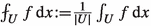as the average of f in U in the integral sense. For functions $$f,g:U\rightarrow \mathbb {R}$$, $$f\lesssim g$$ or $$f\approx g$$ (in U) mean that there exists $$C\geqslant 1$$ such that $$f(y)\leqslant C g(y)$$ or $$C^{-1} f(y)\leqslant g(y)\leqslant C f(y)$$, respectively, for all $$y\in U$$. By $$D=D_x$$ we denote the derivative with respect to the space-variable x.

For $$\varphi :\Omega \times [0,\infty ) \rightarrow [0,\infty )$$ and $$B_r\subset {\mathbb {R}^n}$$, we write

\begin{aligned} \varphi ^+_{B_r}(z){:=}\sup _{x\in B_r\cap \Omega }\varphi (x,z) \quad \text {and}\quad \varphi ^-_{B_r}(z){:=}\inf _{x\in B_r\cap \Omega }\varphi (x,z). \end{aligned}

The same idea and notation is also used for $$F:\Omega \times \mathbb {R}^N\rightarrow [0,\infty ]$$. If the map $$t\mapsto \varphi (x,t)$$, $$t\geqslant 0$$, is increasing for every $$x\in \Omega$$, then the (left-continuous) inverse function with respect to t is defined by

\begin{aligned} \varphi ^{-1}(x,t){:=} \inf \{\tau \geqslant 0: \varphi (x,\tau )\geqslant t\}. \end{aligned}

If $$\varphi$$ is strictly increasing and continuous in t, then this is just the normal inverse function.

### Definition 2.1

We define some conditions for $$\varphi :\Omega \times [0,\infty )\rightarrow [0,\infty )$$ and $$\gamma \in \mathbb {R}$$ related to regularity with respect to the second variable, which are supposed to hold for all $$x\in \Omega$$ and a constant $$L\geqslant 1$$ independent of x. Then,

• (aInc)$$_\gamma$$ $$t\mapsto \varphi (x,t)/t^\gamma$$ is almost increasing on $$(0,\infty )$$ with constant $$L\geqslant 1$$.

• (Inc)$$_\gamma$$ $$t\mapsto \varphi (x,t)/t^\gamma$$ is increasing on $$(0,\infty )$$.

• (aDec)$$_\gamma$$ $$t\mapsto \varphi (x,t)/t^\gamma$$ is almost decreasing on $$(0,\infty )$$ with constant $$L\geqslant 1$$.

• (Dec)$$_\gamma$$ $$t\mapsto \varphi (x,t)/t^\gamma$$ is decreasing on $$(0,\infty )$$.

• (A0) $$L^{-1}\leqslant \varphi (x,1)\leqslant L$$.

Note that this version of (A0) is slightly stronger than the one used in , but they are equivalent under the doubling assumption (aDec), which means that (aDec)$$_q$$ holds for some $$q\geqslant 1$$. We may rewrite (aInc)$$_{p}$$ or (aDec)$$_q$$ ($$p,q>0$$) with constant $$L\geqslant 1$$, as

\begin{aligned}&\varphi (x,ct)\leqslant Lc^p\varphi (x,t)\quad \text {and}\quad L^{-1}C^p \varphi (x,t)\leqslant \varphi (x,Ct)\\&\quad \text {or}\quad L^{-1}c^q \varphi (x,t) \leqslant \varphi (x,ct) \quad \text {and}\quad \varphi (x,Ct) \leqslant L C^q \varphi (x,t), \quad \text {respectively}, \end{aligned}

for all $$(x,t)\in \Omega \times (0,\infty )$$ and $$0<c\leqslant 1 \leqslant C$$. We will use the above inequalities many times without mention. Moreover, if $$\varphi (x,\cdot )\in C^1([0,\infty ))$$ for each $$x\in \Omega$$, then for $$0<p \leqslant q$$

\begin{aligned} \varphi \text{ satisfies } (Inc)_{p} \text{ and } (Dec)_{q} \Longleftrightarrow p \leqslant \frac{t\varphi '(x,t)}{\varphi (x,t)} \leqslant q \ \text{ for } \text{ all } x\in \Omega \text{ and } t\in (0,\infty ). \end{aligned}

We next introduce classes of $$\Phi$$-functions and generalized Orlicz spaces. We refer to  for more details about the basics. In the sequel we omit the words “generalized” and “weak” mentioned in parentheses.

### Definition 2.2

Let $$\varphi :\Omega \times [0,\infty )\rightarrow [0,\infty ]$$. We call $$\varphi$$ a (generalized) $$\Phi$$-prefunction if $$x\mapsto \varphi (x,|f(x)|)$$ is measurable for every measurable function f on $$\Omega$$, and $$t\mapsto \varphi (x,t)$$ is increasing for every $$x\in \Omega$$ and satisfies that $$\varphi (x,0)=\lim _{t\rightarrow 0^+}\varphi (x,t)=0$$ and $$\lim _{t\rightarrow \infty }\varphi (x,t)=\infty$$ for every $$x\in \Omega$$. A $$\Phi$$-prefunction $$\varphi$$ is a

1. (1)

(generalized weak) $$\Phi$$-function, denoted $$\varphi \in {\Phi _{\mathrm {w}}}(\Omega )$$, if it satisfies (aInc)$$_{1}$$;

2. (2)

(generalized) convex $$\Phi$$-function, denoted $$\varphi \in {\Phi _{\mathrm {c}}}(\Omega )$$, if $$t\mapsto \varphi (x,t)$$ is left-continuous and convex for every $$x\in \Omega$$.

If $$\varphi$$ is independent of x, then we write $$\varphi \in {\Phi _{\mathrm {w}}}$$ or $$\varphi \in {\Phi _{\mathrm {c}}}$$ without “$$(\Omega )$$”.

We note that convexity implies (Inc)$$_{1}$$ hence $${\Phi _{\mathrm {c}}}(\Omega )\subset {\Phi _{\mathrm {w}}}(\Omega )$$. While we are mainly interested in convex functions for minimization problems and related PDEs, the class $${\Phi _{\mathrm {w}}}(\Omega )$$ is very useful for constructing approximating functionals, as it allows much more flexibility. This will be utilized several times in this article.

We state some properties of $$\Phi$$-functions, for which we refer to [22, Chapter 2]. We note that in , $$\varphi \simeq \psi$$ for $$\Phi$$-prefunctions $$\varphi$$ and $$\psi$$ means that there exists $$C\geqslant 1$$ such that $$\varphi (x,C^{-1}t)\leqslant \psi (x,t)\leqslant \varphi (x,Ct)$$ for all $$x\in \Omega$$ and $$t\in [0,\infty )$$. This is weaker than $$\approx$$. However, if $$\varphi$$ and $$\psi$$ satisfy (aDec), then $$\simeq$$ and $$\approx$$ are equivalent.

Suppose $$\varphi ,\psi : [0,\infty )\rightarrow [0,\infty )$$ are $$\Phi$$-prefunctions, $$\varphi$$ satisfies (aInc)$$_{1}$$, and $$\psi$$ satisfies (aDec)$$_{1}$$. Then there exist a convex $$\Phi$$-prefunction $$\tilde{\varphi }$$ and a concave $$\Phi$$-prefunction $$\tilde{\psi }$$ such that $$\varphi \approx \tilde{\varphi }$$ and $$\psi \approx \tilde{\psi }$$. Therefore, by Jensen’s inequality we have that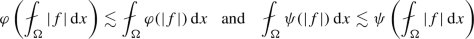for every $$f\in L^1(\Omega )$$, where the implicit constants depend on the constants from the equivalence relations and L from (aInc)$$_1$$ or (aDec)$$_1$$.

We define the conjugate function of $$\varphi :[0,\infty )\rightarrow [0,\infty )$$ by

\begin{aligned} \varphi ^*(x,t) {:=}\sup _{s\geqslant 0} \, (st-\varphi (x,s)). \end{aligned}

The definition directly implies that

\begin{aligned} ts\leqslant \varphi (x,t)+\varphi ^*(x, s)\quad \text {for all }\ s,t\geqslant 0. \end{aligned}

Furthermore, if $$\varphi$$ satisfies (aInc)$$_{p}$$ and (aDec)$$_q$$ for some $$1<p\leqslant q$$, then $$\varphi ^*$$ satisfies (aInc)$$_{q'}$$ and (aDec)$$_{p'}$$ with $$p'=\frac{p}{p-1}$$ and $$q'=\frac{q}{p-1}$$, and for any $$s,t\geqslant 0$$ and $$\varepsilon \in (0,1)$$,

\begin{aligned} ts \leqslant \varphi (x,\varepsilon ^{\frac{1}{p}}t)+\varphi ^*(x,\varepsilon ^{-\frac{1}{p}}s) \lesssim \varepsilon \varphi (x,t)+\varepsilon ^{-\frac{1}{p-1}} \varphi ^*(x,s) \end{aligned}

and

\begin{aligned} ts \leqslant \varphi (x,\varepsilon ^{-\frac{1}{q'}}t)+\varphi ^*(x,\varepsilon ^{\frac{1}{q'}}s) \lesssim \varepsilon ^{-(q-1)} \varphi (x,t)+\varepsilon \varphi ^*(x,s). \end{aligned}

We will refer to any of the previous three formulas as “Young’s inequality”. We also note that $$(\varphi ^*)^*=\varphi$$ if $$\varphi \in {\Phi _{\mathrm {c}}}(\Omega )$$ by [15, Theorem 2.2.6].

If $$\varphi \in {\Phi _{\mathrm {c}}}(\Omega )$$, then there exists $$\varphi '$$, which is increasing and right-continuous, with

\begin{aligned} \varphi (x,t)=\int _0^t \varphi '(x,s)\, \mathrm{d}s. \end{aligned}

We collect some results about this (right-)derivative $$\varphi '$$.

### Proposition 2.3

[Proposition 3.6, ] Let $$\gamma >0$$ and suppose that $$\varphi \in {\Phi _{\mathrm {c}}}(\Omega )$$ has derivative $$\varphi '$$.

1. (1)

If the derivative $$\varphi '$$ satisfies (aInc)$$_{\gamma }$$, (aDec)$$_{\gamma }$$, (Inc)$$_{\gamma }$$ or (Dec)$$_{\gamma }$$, then the function $$\varphi$$ satisfies (aInc)$$_{\gamma +1}$$, (aDec)$$_{\gamma +1}$$, (Inc)$$_{\gamma +1}$$ or (Dec)$$_{\gamma +1}$$, respectively, with the same constant $$L\geqslant 1$$.

2. (2)

If $$\varphi$$ satisfies (aDec)$$_{\gamma }$$, then $$\varphi (x,t)\approx t \varphi '(x,t)$$.

3. (3)

If $$\varphi '$$ satisfies (A0) and (aDec)$$_{\gamma }$$ with constant $$L\geqslant 1$$, then $$\varphi$$ also satisfies (A0), with constant depending on L and $$\gamma$$.

4. (4)

$$\varphi ^*(x,\varphi '(x,t))\leqslant t\varphi '(x,t)$$.

We will use the following inequality for $$C^1$$-regular $$\Phi$$-functions:

### Proposition 2.4

(Proposition 3.8, ) Let $$\varphi \in {\Phi _{\mathrm {c}}}\cap C^1([0,\infty ))$$ with $$\varphi '$$ satisfying (Inc)$$_{p-1}$$ and (Dec)$$_{q-1}$$ for some $$1<p\leqslant q$$. Then, for $$\kappa \in (0,\infty )$$ and $$x,y\in \mathbb {R}^N$$,

\begin{aligned} \varphi (|x-y|) \lesssim \kappa \left[ \varphi (|x|)+\varphi (|y|)\right] + \kappa ^{-1}\frac{\varphi '(|x|+|y|)}{|x|+|y|}|x-y|^2. \end{aligned}

Let $$L^0(\Omega )$$ is the set of the measurable functions on $$\Omega$$. For $$\varphi \in {\Phi _{\mathrm {w}}}(\Omega )$$, the generalized Orlicz space (also known as the Musielak–Orlicz space) is defined by

\begin{aligned} L^{\varphi }(\Omega ){:=}\big \{f\in L^0(\Omega ):\Vert f\Vert _{L^\varphi (\Omega )}<\infty \big \}, \end{aligned}

with the (Luxemburg) norm

\begin{aligned} \Vert f\Vert _{L^\varphi (\Omega )}{:=}\inf \bigg \{\lambda >0: \varrho _{\varphi }\Big (\frac{f}{\lambda }\Big )\leqslant 1\bigg \}, \ \ \text {where}\ \ \varrho _{\varphi }(f){:=}\int _\Omega \varphi (x,|f(x)|)\,\mathrm{d}x. \end{aligned}

We denote by $$W^{1,\varphi }(\Omega )$$ the set of $$f\in L^{\varphi }(\Omega )\cap W^{1,1}(\Omega )$$ satisfying that $$|Df| \in L^{\varphi }(\Omega )$$ with the norm $$\Vert f\Vert _{W^{1,\varphi }(\Omega )}{:=}\Vert f\Vert _{L^\varphi (\Omega )}+\Vert \,|Df|\Vert _{L^\varphi (\Omega )}$$. Note that if $$\varphi$$ satisfies (aDec)$$_q$$ for some $$q\geqslant 1$$, then $$f\in L^\varphi (\Omega )$$ if and only if $$\varrho _\varphi (f)<\infty$$, and if $$\varphi$$ satisfies (A0), (aInc)$$_{p}$$ and (aDec)$$_q$$ for some $$1<p\leqslant q$$, then $$L^\varphi (\Omega )$$ and $$W^{1,\varphi }(\Omega )$$ are reflexive Banach spaces. In addition, we denote by $$W^{1,\varphi }_0(\Omega )$$ the closure of $$C^\infty _0(\Omega )$$ in $$W^{1,\varphi }(\Omega )$$. For more information about the generalized Orlicz and Orlicz–Sobolev spaces, we refer to the monographs [7, 22, 30] and also [15, Chapter 2].

### 2.2 New conditions

The condition (A1) was introduced in  (see also ) and is essentially optimal for the boundedness of the maximal operator in generalized Orlicz spaces. It also implies the Hölder continuity of solutions and (quasi)minimizers [5, 24, 25]. For higher regularity, we introduced in  a “vanishing (A1)” condition, denoted (VA1), and a weak vanishing version, (wVA1). These previous studies applied to $$\Phi$$-functions $$\varphi :\Omega \times [0,\infty )\rightarrow [0,\infty )$$ and discussed the sharpness of the conditions.

We generalize the conditions to the non-Uhlenbeck situation. It can be easily seen that (VA1)$$\Longrightarrow$$(wVA1)$$\Longrightarrow$$(A1). The results of this paper require only (wVA1), but (VA1) is included since it is simpler to check and is a sufficient condition.

### Definition 2.5

Let $$G:\Omega \times {\mathbb {R}^n} \rightarrow \mathbb {R}^N$$, $$N\in \mathbb N$$, $$\varepsilon \in [0,1]$$, $$K, \bar{L}>0$$, $$r\in (0,1]$$ and $$\omega :[0,1]\rightarrow [0,1]$$. We consider the inequality

\begin{aligned} |G(x,\xi )-G(y,\xi )|\leqslant \bar{L}\omega (r)\big (|G(y,\xi )|+1\big ) \quad \text {when }\ |G(y,\xi )|\in [0,K|B_r|^{-1+\varepsilon }] \end{aligned}

for all $$x,y\in B_r\cap \Omega$$ and $$\xi \in {\mathbb {R}^n}$$. We say that G satisfies:

1. (A1)

if for any $$K>0$$ there exists $$\bar{L}=\bar{L}_K>0$$ such that the inequality holds for $$\omega \equiv 1$$ and $$\varepsilon =0$$.

2. (VA1)

if for any $$K>0$$ there exists $$\bar{L}=\bar{L}_K>0$$ and there exists a modulus of continuity $$\omega :[0,1]\rightarrow [0,1]$$ such that the inequality holds for $$\varepsilon =0$$.

3. (wVA1)

if for any $$K>0$$ there exists $$\bar{L}=\bar{L}_K>0$$ and for every $$\varepsilon >0$$ there exists a modulus of continuity $$\omega =\omega _\varepsilon :[0,1]\rightarrow [0,1]$$ such that the inequality holds.

Here, a modulus of continuity $$\omega$$ means that $$\omega$$ is concave and nondecreasing and satisfies $$\lim _{r\rightarrow 0^+}\omega (r)=\omega (0)=0$$.

If we compare these conditions in the case $$N=1$$ with the previously mentioned conditions in earlier papers, we see that they do not look exactly the same. First of all the earlier conditions correspond to the case $$K=1$$ only. If we consider quasi-convex domains $$\Omega$$ and $$\varphi$$ satisfies (aDec), then the condition with any K is equivalent to the condition with fixed K. This is proved by a simple chain argument, see [23, Lemma 3.3] for (A1).

Furthermore, the earlier conditions did not allow $$\xi$$ satisfying $$|G(y,\xi )|<\omega (r)$$. For instance, the old formulation of (VA1) (for $$G(x,\xi )=\varphi (x,|\xi |)$$) was to assume that

\begin{aligned} |\varphi (x,t)-\varphi (y,t)|\leqslant L \omega (r) \varphi (y,t) \quad \text {when } \varphi (y,t)\in [\omega (r), |B_r|^{-1}] \end{aligned}

Compared to new (VA1), the right-hand side has $$\varphi (y,t)$$ instead of $$\varphi (y,t)+1$$ but on the other hand the inequality is not assumed for $$\varphi (y,t)\in [0,\omega (r))$$. However, we can show that these are again equivalent if $$\varphi \in {\Phi _{\mathrm {w}}}(\Omega )$$ is continuous in t. A similar argument applies to the other conditions as well.

Let us show that the old version of (VA1) implies (VA1) with the same $$\omega$$. Indeed, we need only show that the old condition implies (VA1) when $$\varphi (y,t)<\omega (r)$$ since this is trivial when $$\varphi (y,t)\in [\omega (r), |B_r|^{-1}]$$. Fix $$B_r$$ and let $$y\in B_r$$. Since $$\varphi$$ is increasing and continuous, we can find $$s>t$$ with $$\varphi (y,s)=\omega (r)$$. Then by the old condition,

\begin{aligned} \varphi (x,t)\leqslant \varphi (x,s)\leqslant (1+L \omega (r)) \varphi (y,s) \leqslant (L+1)\omega (r) \quad \text {for all }\ x\in B_r. \end{aligned}

Hence we obtain (VA1) as follows:

\begin{aligned} |\varphi (x,t)-\varphi (y,t)|&\leqslant \varphi (x,s)+\varphi (y,s)\leqslant (L+2)\omega (r)\\&\leqslant (L+2)\omega (r)(\varphi (y,t)+1). \end{aligned}

For the opposite implication, if $$\varphi (y,t)\in [\omega (r)^\frac{1}{2}, |B_r|^{-1}]$$, then

\begin{aligned} \omega (r) (\varphi (y,t) + 1) \leqslant 2 \omega (r)^\frac{1}{2}\varphi (y,t). \end{aligned}

It follows that (VA1) with $$\omega$$ implies the old version of (VA1) with $$\omega ^{\frac{1}{2}}$$ in place of $$\omega$$.

In summary, under minimal and natural assumptions on G, our conditions are equivalent to previous versions. In particular, from [22, Chapter 7] and [28, Sect. 8], we know that (A1) is equivalent to logarithmic Hölder continuity in the variable exponent case $$\varphi (x,t)=t^{p(x)}$$ while (VA1) and (wVA1) hold with $$\omega$$ if and only if $$p\in C^{0,\omega }$$. For the double phase case $$\varphi (x,t)=t^p + a(x)t^q$$, (VA1) is more or less equivalent to $$\frac{q}{p} < 1+\frac{\alpha }{n}$$ while (wVA1) and (A1) require only $$\frac{q}{p} \leqslant 1+\frac{\alpha }{n}$$, where $$a\in C^{0,\alpha }(\Omega )$$.

### Remark 2.6

Baroni, Colombo and Mingione [2, 9] initiated the study of double phase with additional information on the minimizer. Specifically, they considered bounded or Hölder continuous minimizers and showed that the assumption $$\frac{q}{p} \leqslant 1+\frac{\alpha }{n}$$ can be relaxed in these cases. Ok  considered analogous results with Lebesgue integrability information on the minimizer. In [5, 24], the generalized Orlicz case with additional information was considered, but only for lower regularity, i.e. Harnack’s inequality and Hölder continuity for some $$\gamma >0$$. It is possible, but non-trivial, to develop the ideas from this paper to prove maximal regularity in the generalized Orlicz case with additional information; we will return to this issue in a follow-up paper .

## 3 Construction of Growth Function

In this section, we construct an auxiliary function $$\varphi \in {\Phi _{\mathrm {c}}}(\Omega )$$ that measures the growth of $$A:\Omega \times {\mathbb {R}^n} \rightarrow {\mathbb {R}^n}$$ or $$F:\Omega \times {\mathbb {R}^n} \rightarrow [0,\infty )$$.

### Definition 3.1

Let $$A:\Omega \times {\mathbb {R}^n} \rightarrow {\mathbb {R}^n}$$ satisfy Assumption 1.1(1). We say that $$\varphi \in {\Phi _{\mathrm {c}}}(\Omega )$$ is a growth function of A if the following conditions are satisfied: $$\varphi (x,\cdot )\in C^1([0,\infty ))$$ for every $$x\in \Omega$$; $$\varphi '$$ satisfies (A0), (Inc)$$_{p_1-1}$$ and (Dec)$${_{q_1}-1}$$ for some $$1<p_1\leqslant q_1$$; and

\begin{aligned}&|A(x,\xi )|+ |\xi |\,|D_\xi A(x,\xi )|\leqslant \Lambda \varphi '(x,|\xi |), \end{aligned}
(3.1)
\begin{aligned}&D_\xi A(x,\xi )\tilde{\xi }\cdot \tilde{\xi }\geqslant \nu \frac{\varphi '(x,|\xi |)}{|\xi |}|\tilde{\xi }|^2 \end{aligned}
(3.2)

for some $$0<\nu \leqslant \Lambda$$ and for all $$x\in \Omega$$ and $$\xi , \tilde{\xi }\in {\mathbb {R}^n} \setminus \{ 0\}$$.

Let $$F:\Omega \times \mathbb {R}\rightarrow \mathbb {R}$$ satisfy Assumption 1.5(1). We say that $$\varphi \in {\Phi _{\mathrm {c}}}(\Omega )$$ is a growth function of F if it is a growth function of $$A=D_\xi F$$ in the above sense.

### Remark 3.2

In the above definition, we may assume without loss of generality that $$\varphi (x,\cdot )\in C^2((0,\infty ))$$. Indeed, define $$\psi '(x,t){:=}\int _0^t\frac{\varphi '(x,s)}{s}\,\mathrm{d}s$$ and $$\psi (x,t){:=}\int _0^t\psi '(x,s)\,\mathrm{d}s$$. Then $$\psi (x,\cdot )\in C^2((0,\infty ))$$ satisfies $$\varphi \approx \psi$$, $$\min \{1,p_1-1\}\varphi '(x,t)\leqslant \psi '(x,t) \leqslant \max \{1,q_1-1\} \varphi '(x,t)$$, and (Inc)$$_{p_1-1}$$ and (Dec)$${_{q_1}-1}$$ with the same $$p_1$$ and $$q_1$$.

### Proposition 3.3

Every $$A:\Omega \times {\mathbb {R}^n} \rightarrow {\mathbb {R}^n}$$ satisfying Assumption 1.1 has a growth function $$\varphi \in {\Phi _{\mathrm {c}}}(\Omega )$$ with constants $$1< p_1\leqslant q_1$$ and $$0<\nu \leqslant \Lambda$$ depending only on the parameters p, q and L in Assumption 1.1. Specifically, $$p_1=p$$ and $$q_1\geqslant q$$.

### Proof

Define $$\psi \in {\Phi _{\mathrm {w}}}(\Omega )$$ by

\begin{aligned} \psi (x,t){:=}\int _0^t\psi '(x,s)\,\mathrm{d}s \quad \text {and}\quad \psi '(x, t) {:=} \sup _{|\xi |=t} |\xi |\,|D_\xi A(x,\xi )|. \end{aligned}

By Assumption 1.1(2), $$\psi '$$ satisfies (A0), (aInc)$$_{p-1}$$ and (aDec)$$_{q-1}$$, and hence $$\psi$$ satisfies (A0), (aInc)$$_{p}$$ and (aDec)$$_q$$ (Proposition 2.3(1)). In view of [22, Lemmas 2.2.1 and 2.2.6], there exists $$\widetilde{\psi }\in {\Phi _{\mathrm {c}}}(\Omega )$$ with $$\widetilde{\psi }\approx \psi$$ which satisfies (A0), (Inc)$$_{p}$$ and (Dec)$$_{q_1}$$ for some $$q_1\geqslant q$$. Next we define $$\varphi \in {\Phi _{\mathrm {c}}}(\Omega )$$ with $$\varphi (x,\cdot ) \in C^1([0,\infty ))$$ for every $$x\in \Omega$$ by

\begin{aligned} \varphi (x,t){:=}\int _0^t \frac{\widetilde{\psi }(x,s)}{s}\,\mathrm{d}s. \end{aligned}

Then $$\varphi '(x,t)= \frac{\widetilde{\psi }(x,t)}{t} \approx \frac{\psi (x,t)}{t}\approx \psi '(x,t)$$ and $$\varphi '$$ satisfies (A0), (Inc)$$_{p-1}$$ and (Dec)$${_{q_1}-1}$$.

We now show that $$\varphi$$ is the desired growth function. Note that by Assumption 1.1(2),

\begin{aligned}\begin{aligned} |A(x,\xi )|&=\left| \int _0^1\frac{\mathrm{d}}{\mathrm{d}t} A (x,t\xi ) \,\mathrm{d}t\right| =\left| \int _0^1 D_\xi A(x,t\xi )\cdot \xi \,\mathrm{d}t\right| \\&\leqslant \int _0^1 |D_\xi A(x,t\xi )|\,|\xi | \,\mathrm{d}t \\&\leqslant L \left( \int _0^1 t^{p-2} \,\mathrm{d}t\right) |D_\xi A(x,\xi )|\,|\xi | \leqslant \frac{L}{p-1} \psi '(x,|\xi |). \end{aligned} \end{aligned}

Then (3.1) follows from $$\varphi '\approx \psi '$$ and the definition of $$\psi '$$, and (3.2) holds by Assumption 1.1(3) with $$\varphi '\approx \psi '$$ and the definition of $$\psi '$$.

From now on, if $$A:\Omega \times {\mathbb {R}^n} \rightarrow {\mathbb {R}^n}$$ satisfies Assumption 1.1, we take its growth function as the one constructed in the above proposition, hence the constants $$p_1$$, $$q_1$$, $$\nu$$, $$\Lambda$$ in Definition 3.1 depend on the constants p, q, L in Assumption 1.1.

### Remark 3.4

Inequality (3.2) with $$\varphi '$$ satisfying (Inc)$$_{p-1}$$ and (Dec)$${_{q_1}-1}$$ implies the following strict monotonicity condition:

\begin{aligned} (A(x,\xi )-A(x,\tilde{\xi })) \cdot (\xi -\tilde{\xi }) \gtrsim \frac{\varphi '(x,|\xi |+|\tilde{\xi }|)}{|\xi |+|\tilde{\xi }|}|\xi - \tilde{\xi }|^2, \qquad x\in \Omega , \ \ \xi ,\tilde{\xi }\in {\mathbb {R}^n} \setminus \{0\}. \end{aligned}
(3.3)

Furthermore, letting $$\tilde{\xi }\rightarrow 0$$ in the preceding inequality, we also have the coercivity condition

\begin{aligned} A(x,\xi ) \cdot \xi \gtrsim \varphi '(x,|\xi |)|\xi | \approx \varphi (x,|\xi |), \qquad x\in \Omega , \ \ \xi \in {\mathbb {R}^n} , \end{aligned}

which yields that

\begin{aligned} \varphi (x,|\xi |) \lesssim A(x,\xi )\cdot \xi \leqslant |\xi | |A(x,\xi )| \lesssim |\xi |\varphi '(x,|\xi |) \approx \varphi (x,|\xi |), x\in \Omega ,\ \ \xi \in {\mathbb {R}^n} . \end{aligned}
(3.4)

From now on, we denote

\begin{aligned} {A^{(-1)}}(x,\xi ){:=}|\xi |\,A(x,\xi ), \end{aligned}

and write $$\varphi \approx {A^{(-1)}}$$ as an abbreviation of (3.4). (The notation is chosen since $$A^{(-1)}$$ is comparable to the antiderivative of A.) Since (A1) is invariant under equivalence [22, Lemma 4.1.3] we obtain the following result:

### Proposition 3.5

Let $$A:\Omega \times {\mathbb {R}^n} \rightarrow {\mathbb {R}^n}$$ satisfy Assumption 1.1 and $$\varphi$$ be its growth function. Then $${A^{(-1)}}$$ satisfies (A1) if and only if $$\varphi$$ satisfies (A1).

We next consider the continuity hypotheses (wVA1). This assumption is not invariant under equivalence, but we have the following implications for the growth function. The second part of the proposition is a stronger version of (A1), where we notice that the range of $$\varphi ^-_{B_r}(t)$$ is changed to $$[\omega (r),|B_r|^{-1}]$$, which is needed later on. Here $$p'$$ denotes the Hölder conjugate exponent of p, i.e. $$p'=\frac{p}{p-1}$$.

### Proposition 3.6

Let $$A:\Omega \times {\mathbb {R}^n} \rightarrow {\mathbb {R}^n}$$ satisfy Assumption 1.1 and $$\varphi$$ be its growth function. If $${A^{(-1)}}$$ satisfies (wVA1), then for each $$\varepsilon \in (0,1]$$ with $$\omega =\omega _\varepsilon$$ and $$r\in (0,1]$$,

1. (1)

for all $$x,y\in B_r\cap \Omega$$ and $$\xi \in \mathbb {R}^n$$ satisfying $$\varphi _{B_r}^-(|\xi |)\in [0, |B_r|^{-1+\varepsilon }]$$,

\begin{aligned} |A(x,\xi )-A(y,\xi )| \leqslant c\omega (r)^\frac{1}{p'}\big ((\varphi ')^-_{B_r}(|\xi |)+1\big ); \end{aligned}
2. (2)

for all $$t>0$$ satisfying $$\varphi ^-_{B_r}(t)\in [\omega (r),|B_r|^{-1}]$$,

\begin{aligned} \varphi ^+_{B_r}(t) \leqslant c \varphi ^-_{B_r}(t). \end{aligned}

Here the constants $$c>0$$ depend on p, q, L and $$\bar{L}_{2c_1}$$, where $$c_1\geqslant 1$$ is the implicit constant from $$\varphi \approx {A^{(-1)}}$$ (3.4).

### Proof

Suppose that $$\xi \in {\mathbb {R}^n}$$ satisfies $$\varphi _{B_r}^-(|\xi |)\leqslant |B_r|^{-1+\varepsilon }$$. Choose $$x'\in B_r$$ with $$\varphi (x',|\xi |) \leqslant 2\varphi _{B_r}^-(|\xi |)$$ so that $$\varphi (x',|\xi |)\leqslant 2|B_r|^{-1+\varepsilon }$$. This together with $${A^{(-1)}}\approx \varphi$$ (3.4) yields

\begin{aligned} |{A^{(-1)}}(x',\xi )| \leqslant c_1 \varphi (x', |\xi |)\leqslant 2c_1|B_r|^{-1+\varepsilon }. \end{aligned}

Therefore, by (wVA1) with $$K=2c_1$$ we have that for any $$x,y\in B_r$$

\begin{aligned} \begin{aligned}&|{A^{(-1)}}(x,\xi )-{A^{(-1)}}(y,\xi )| \\&\qquad \leqslant |{A^{(-1)}}(x,\xi )-{A^{(-1)}}(x',\xi )|+|{A^{(-1)}}(x',\xi )-{A^{(-1)}}(y,\xi )| \\&\qquad \leqslant 2\bar{L}_{2c_1} \omega (r)(|{A^{(-1)}}(x',\xi )|+1)\\&\qquad \approx \omega (r)\left( \varphi (x',|\xi |)+1\right) \approx \omega (r)( \varphi ^-_{B_r}(|\xi |)+1) \end{aligned} \end{aligned}
(3.5)

and, using $${A^{(-1)}}\approx \varphi$$,

\begin{aligned} \varphi (x,|\xi |)&\lesssim |{A^{(-1)}}(x,\xi )-{A^{(-1)}}(x',\xi )| + \varphi (x',|\xi |)\nonumber \\&\lesssim \omega (r)( \varphi ^-_{B_r}(|\xi |)+1)+\varphi ^-_{B_r}(|\xi |). \end{aligned}
(3.6)

When also $$\varphi _{B_r}^-(|\xi |)\geqslant \omega (r)\in [0,1]$$, we can continue this by

\begin{aligned} \varphi (x,|\xi |) \lesssim \omega (r)( \varphi ^-_{B_r}(|\xi |)+1)+\varphi ^-_{B_r}(|\xi |) \leqslant 2\varphi ^-_{B_r}(|\xi |)+\omega (r) \leqslant 3\varphi ^-_{B_r}(|\xi |). \end{aligned}

Since the implicit constant in the preceding inequality is independent of $$\varepsilon$$, we obtain the inequality in (2) for $$\xi$$ with $$\varphi _{B_r}^-(|\xi |)\in [\omega (r), |B_r|^{-1})$$. Moreover, the continuity of $$\varphi$$ in the t variable implies the same inequality for $$\xi$$ with $$\varphi _{B_r}^-(|\xi |)= |B_r|^{-1}$$. This completes the proof of claim (2).

Still considering $$\varphi _{B_r}^-(|\xi |)\geqslant \omega (r)\in [0,1]$$, we move on to prove (1). Since $$\varphi$$ satisfies (A0) and (Inc)$$_{p}$$, we conclude that $$|\xi |\gtrsim \omega (r)^{1/p}$$. Dividing (3.5) by $$|\xi |$$, we obtain

\begin{aligned} \begin{aligned} |A(x,\xi )-A(y,\xi )| \lesssim \omega (r)( \varphi ^-_{B_r}(|\xi |)+1) |\xi |^{-1}&\leqslant \omega (r)( (\varphi ')^-_{B_r}(|\xi |)+\omega (r)^{-1/p})\\&\leqslant \omega (r)^{\frac{1}{p'}}( (\varphi ')^-_{B_r}(|\xi |)+1), \end{aligned} \end{aligned}

where we used that $$t\varphi '(x,t)\approx \varphi (x,t)$$.

It remains to prove (1) when $$\varphi _{B_r}^-(|\xi |)< \omega (r)$$. By (3.6), it follows that $$\varphi _{B_r}^+(|\xi |)\lesssim \omega (r)$$. Hence applying Proposition 2.3(4), for any $$x\in B_r$$,

\begin{aligned} |A(x,\xi )| \approx \varphi '(x,|\xi |)&= (\varphi ^*)^{-1}(x,\varphi ^*(x,\varphi '(x,|\xi |))) \lesssim (\varphi ^*)^{-1}( x,\omega (r)) \\&\lesssim \omega (r)^{\frac{1}{p'}} \end{aligned}

and so (1) follows in this case also.

Let $$F:\Omega \times {\mathbb {R}^n} \rightarrow [0,\infty )$$ satisfy Assumption 1.5 and $$\varphi \in {\Phi _{\mathrm {c}}}(\Omega )$$ be its growth function. By (3.4) with $$A=D_\xi F$$,

\begin{aligned} F(x,\xi ) = \int _0^1 D_\xi F(x,t\xi )\cdot \xi \,\mathrm{d}x \approx \varphi (x,|\xi |) \quad \text {for all} \ x\in \Omega \hbox { and } \xi \in {\mathbb {R}^n} , \end{aligned}
(3.7)

In the same way as Propositions 3.5 and 3.6, we can also prove the following.

### Proposition 3.7

Let $$F:\Omega \times {\mathbb {R}^n} \rightarrow \mathbb {R}$$ satisfy Assumption 1.5 and $$\varphi$$ be its growth function. Then F satisfies (A1) if and only if $$\varphi$$ satisfies (A1).

If F satisfies (wVA1), then for each $$\varepsilon \in (0,1]$$ with $$\omega =\omega _\varepsilon$$ and $$r\in (0,1]$$,

1. (1)

for all $$\xi \in \mathbb {R}^n$$ satisfying $$\varphi _{B_r}^-(|\xi |)\in [0, |B_r|^{-1+\varepsilon }]$$,

\begin{aligned} F^+_{B_r}(\xi )- F^-_{B_r}(\xi ) \leqslant c \omega (r)\big (\varphi ^-_{B_r}(|\xi |)+1\big ) \end{aligned}
2. (2)

for all $$t>0$$ satisfying $$\varphi ^-_{B_r}(t)\in [\omega (r),|B_r|^{-1}]$$,

\begin{aligned} \varphi ^+_{B_r}(t) \leqslant c \varphi ^-_{B_r}(t). \end{aligned}

Here the constants $$c>0$$ depend on p, q, L and $$\bar{L}_{2c_2}$$, where $$c_2\geqslant 1$$ is the implicit constant from $$\varphi \approx F$$ (3.7).

Finally, we consider the relation between (wVA1) of A and F. Based on this result we can say that the condition (wVA1) is weaker in the minimization case than in the PDE case.

### Proposition 3.8

Let $$F:\Omega \times {\mathbb {R}^n} \rightarrow \mathbb {R}$$ satisfy Assumption 1.5 and set $$A^{(-1)}(x,\xi ){:=}|\xi | D_\xi F(x,\xi )$$. If $${A^{(-1)}}$$ satisfies (wVA1), then so does F with the same $$\omega$$.

### Proof

Let $$\varphi$$ be a growth function of F. Fix any $$B_r\subset {\mathbb {R}^n}$$ with $$r\leqslant 1$$. Suppose $$F^-_{B_r}(\xi )\in [0, K|B_r|^{-1+\varepsilon }]$$. Since $$|{A^{(-1)}}(y,\xi )|\leqslant c_1\varphi (y,|\xi |) \leqslant c_1c_2 F(y,\xi )$$ for all $$\xi \in {\mathbb {R}^n}$$ by $$\varphi \approx {A^{(-1)}}$$ (3.4) and $$\varphi \approx F$$ (3.7) with implicit constants $$c_1$$ and $$c_2$$, respectively, we obtain

\begin{aligned} |{A^{(-1)}}(y,\xi )|\in [0,Kc_1c_2 |B_r|^{-1+\varepsilon }]. \end{aligned}

Thus we can use (wVA1) of $${A^{(-1)}}$$. Define $$e=\frac{\xi }{|\xi |}$$. Then

\begin{aligned}\begin{aligned} F(x,\xi )-F(y,\xi )&= \int _0^{|\xi |} (A(x,te)-A(y,te))\cdot e\, \mathrm{d}t\\&\leqslant \bar{L}_{Kc_1c_2}\omega (r)\int _0^{|\xi |} \big [|A(y,te)|+1\big ]\, \mathrm{d}t \\&\lesssim \omega (r)\int _{0}^{|\xi |} [\varphi '(y,t)+1]\, \mathrm{d}t = \omega (r)(\varphi (y,|\xi |)+|\xi |)\\&\lesssim \omega (r) (\varphi (y,|\xi |)+1)\approx \omega (r) (F(y,\xi )+1) \end{aligned} \end{aligned}

for any $$x,\tilde{x} \in B_r\cap \Omega$$, which is (wVA1) of F.

## 4 Auxiliary Regularity Results

In this section we collect results on two types of approximating problems, namely non-autonomous problems with Uhlenbeck structure and autonomous problems without Uhlenbeck structure.

### 4.1 Quasiminimizers

For $$\psi \in {\Phi _{\mathrm {w}}}(\Omega )$$, we say that $$u\in W^{1,\psi }_{\mathrm {loc}}(\Omega )$$ is a local $$\psi$$-quasiminimizer if there exists $$Q\geqslant 1$$ such that

\begin{aligned} \int _{\mathrm{supp}\,(u-v)} \psi (x,|Du|)\,\mathrm{d}x \leqslant Q \int _{\mathrm{supp}\,(u-v)} \psi (x,|Dv|)\,\mathrm{d}x \end{aligned}

for all $$v\in W^{1,\psi }_{\mathrm {loc}}(\Omega )$$ with $$\mathrm{supp}\,(u-v)\Subset \Omega$$. Quasiminimizers of energy functionals with generalized Orlicz growth have been studied e.g. in [5, 6, 24, 26].

Let $$A:\Omega \times {\mathbb {R}^n} \rightarrow {\mathbb {R}^n}$$ satisfy Assumption 1.1 and $$\varphi$$ be its growth function. Let $$u\in W^{1,1}_{\mathrm {loc}}(\Omega )$$ be a local weak solution to ($$\mathrm {div}A$$). By $$\varphi \approx {A^{(-1)}}$$ (3.4) and the assumption $$|{A^{(-1)}}(\cdot ,Du)| \in L^1_{\mathrm {loc}}(\Omega )$$ we see that $$u \in W^{1,\varphi }_{\mathrm {loc}}(\Omega )$$. Assuming density, we obtain by approximation that

\begin{aligned} \int _\Omega A(x,Du)\cdot D\zeta \,\mathrm{d}x =0 \quad \text {for every }\ \zeta \in W^{1,\varphi }(\Omega ) \ \text { with }\ \mathrm {supp}\,(\zeta )\Subset \Omega . \end{aligned}

Using $$\varphi \approx {A^{(-1)}}$$, the identity above with $$\zeta =u-v$$, Young’s inequality and Proposition 2.3(4), we have

\begin{aligned} \begin{aligned} \int _{\mathrm {supp}\,(u-v)} \varphi (x,|Du|)\,\mathrm {d}x&\lesssim \int _{\mathrm {supp}\,(u-v)} A(x,Du)\cdot Du\,\mathrm {d}x\\ {}&=\int _{\mathrm {supp}\,(u-v)} A(x,Du)\cdot Dv\,\mathrm {d}x \\ {}&\lesssim \int _{\mathrm {supp}\,(u-v)} \frac{\varphi (x,|Du|)}{|Du|} |Dv| \,\mathrm {d}x \\ {}&\lesssim \varepsilon \int _{\mathrm {supp}\,(u-v)} \varphi (x,|Du|)\,\mathrm {d}x \\ {}&\qquad +\varepsilon ^{1-q}\int _{\mathrm {supp}\,(u-v)} \varphi (x,|Dv|)\,\mathrm {d}x \end{aligned} \end{aligned}

for every $$\varepsilon \in (0,1]$$ and $$v\in W^{1,\varphi }(\Omega )$$ with $$\mathrm{supp}\,(u-v) \Subset \Omega$$. Thus

\begin{aligned} \int _{\mathrm{supp}\,(u-v)} \varphi (x,|Du|)\,\mathrm{d}x \leqslant Q \int _{\mathrm{supp}\,(u-v)} \varphi (x,|Dv|)\,\mathrm{d}x \end{aligned}

for some $$Q\geqslant 1$$ depending only on p, q and L so that u is a $$\varphi$$-quasiminimizer. Note that the density of smooth functions holds if $$\varphi$$ satisfies (A0), (A1) and (aDec) [22, Theorem 6.4.7].

Thus we can apply the regularity results for quasiminimizers in  to a local weak solution to ($$\mathrm {div}A$$). In particular, we have the following Hölder regularity and higher integrability results for ($$\mathrm {div}A$$). For the last estimate, we refer to [28, Theorem 4.7].

### Theorem 4.1

 Let $$A:\Omega \times {\mathbb {R}^n} \rightarrow {\mathbb {R}^n}$$ satisfy Assumption 1.1 with constants $$L\geqslant 1$$ and $$1<p \leqslant q$$ and have growth function $$\varphi \in {\Phi _{\mathrm {c}}}(\Omega )$$ and let $$u\in W^{1,1}_\mathrm {loc}(\Omega )$$ be a local weak solution to ($$\mathrm {div}A$$). If $$A^{(-1)}$$ satisfies (A1) with constant $$\bar{L}_K>0$$, then

• $$u\in C^{\alpha }_{\mathrm {loc}}(\Omega )$$ for some $$\alpha =\alpha (n,p,q,L,\bar{L}_1)\in (0,1)$$;

• $$A^{(-1)}(\cdot , Du)\approx \varphi (\cdot ,|Du|)\in L^{1+\sigma }_{\mathrm {loc}}(\Omega )$$ for some $$\sigma =\sigma (n,p,q,L,\bar{L}_1)>0$$. Moreover, for some $$c=c(n,p,q,L,\bar{L}_1)>0$$ we have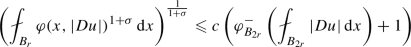whenever $$B_{2r}\Subset \Omega$$ with $$|B_{2r}|\leqslant 1$$ and $$\Vert Du\Vert _{L^\varphi (B_{2r})}\leqslant 1$$.

### Remark 4.2

The above theorem still holds in the setting of the minimization problem as in Theorem 1.6, where we assume that F satisfies (A1).

### Remark 4.3

Assumptions 1.1 and 1.5 involve the derivative of A or the second derivative of F. In fact, these are only needed for the maximal regularity. For the previous theorem, Assumptions 1.1 could be replaced by weaker assumptions, such as

1. (1)

For every $$x\in \Omega$$, $$A(x, 0)= 0$$, $$A(x,\cdot )\in C^{0}(\mathbb {R}^n\setminus \{ 0\}; \mathbb {R}^n)$$ and for every $$\xi \in \mathbb {R}^n$$, $$A(\cdot ,\xi )$$ is measurable.

2. (2)

There exist $$L\geqslant 1$$ and $$1<p<q$$ such that the radial function $$t\mapsto |A(x,te)|$$ satisfies (A0), (aInc)$$_{p-1}$$ and (aDec)$$_{q-1}$$ for every $$x\in \Omega$$ and $$e\in {\mathbb {R}^n}$$ with $$|e|=1$$.

3. (3)

There exists $$L\geqslant 1$$ such that

\begin{aligned} |\xi '|\,| A(x,\xi ')| \leqslant L\, A(x,\xi ) \cdot \xi \end{aligned}

for all $$x\in \Omega$$ and $$\xi ,\xi ' \in {\mathbb {R}^n}$$ with $$|\xi |=|\xi '|$$.

In this case the growth function $$\varphi$$ is replaced by

\begin{aligned} \psi (x,t){:=} \sup _{|\xi |=t}|\xi |\,|A(x,\xi )|. \end{aligned}

### 4.2 Regularity results for autonomous Problems

We consider Assumption 1.1 for an autonomous function $${\bar{A}}:{\mathbb {R}^n} \rightarrow {\mathbb {R}^n}$$ via its trivial extension $${\bar{A}}(x,\xi ){:=} {\bar{A}}(\xi )$$. We may apply Proposition 3.3 to conclude that such $${\bar{A}}$$ has a growth function $${\bar{\varphi }}\in {\Phi _{\mathrm {c}}}(\Omega )$$. An inspection of the proof of Proposition 3.3 shows that this $${\bar{\varphi }}$$ can be chosen autonomous as well, and we will assume hereafter that $${\bar{\varphi }}\in {\Phi _{\mathrm {c}}}$$, i.e., $${\bar{\varphi }}= {\bar{\varphi }}(t)$$. Moreover, in view of Remark 3.2, we also assume that $${\bar{\varphi }}\in C^1([0,\infty ))\cap C^2((0,\infty ))$$.

With these $${\bar{A}}$$ and $${\bar{\varphi }}$$, we present regularity results of weak solutions to the following autonomous problem

The following results are variations of known regularity results for equations with Orlicz growth, and the proofs are similar to previous ones. In particular, in the Uhlenbeck case $${\bar{A}}(\xi )=\frac{{\bar{\varphi }}'(|\xi |)}{\xi }\xi$$, we considered them in . Therefore, we outline the proofs and point out differences compared with the references. The first result is local $$C^{1,\alpha }$$-regularity.

### Lemma 4.4

Let $${\bar{A}}:\mathbb {R}^n\rightarrow \mathbb {R}^n$$ satisfy Assumption 1.1 with $$A(x,\xi )\equiv {\bar{A}}(\xi )$$ and constants $$L\geqslant 1$$ and $$1<p\leqslant q$$, and $${\bar{\varphi }}\in {\Phi _{\mathrm {c}}}$$ be its growth function. If $${\bar{u}}{}\in W^{1,{\bar{\varphi }}}(B_r)$$ is a weak solution to ($$\mathrm {div}{\bar{A}}$$), then $$D{\bar{u}}\in C^{0,\bar{\alpha }}_{\mathrm {loc}}(B_r,{\mathbb {R}^n} )$$ for some $$\bar{\alpha }\in (0,1)$$ with the following estimates: for any $$B_\rho \subset B_r$$ and any $$\tau \in (0,1)$$,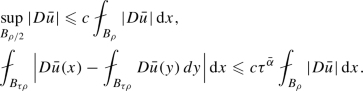Here $$\bar{\alpha }\in (0,1)$$ and $$c>0$$ depend only on the constants n, p, q and L.

### Outline of proof

The proof is almost the same as for the Uhlenbeck case, that is [28, Lemma 4.12] with a proof in [28, Appendix A]. We also refer to  for the case $${\bar{\varphi }}(t)=t^p$$. We only need to slightly modify the beginning and approximation parts to the general case $${\bar{A}}(\xi )$$.

Instead of the equation ($$\mathrm {div}{\bar{A}}$$), we consider approximate non-degenerate equations. For $$0<\varepsilon <\frac{1}{2}$$, define

\begin{aligned} {\bar{A}}_\varepsilon (\xi ){:=} {\bar{A}}\big ( (\tfrac{\varepsilon }{|\xi |}+1)\xi \big )\frac{|\xi |}{\varepsilon +|\xi |} \quad \text {and}\quad {\bar{\varphi }}_\varepsilon ' (t){:=} \frac{{\bar{\varphi }}'(\varepsilon +t)}{\varepsilon +t}t. \end{aligned}

Note that $${\bar{\varphi }}_\varepsilon (t) {:=} \int _0^t{\bar{\varphi }}'_\varepsilon (s)\,\mathrm{d}s$$ is exactly the same as $$\varphi _\varepsilon (t)$$ in [28, (A.1)], hence, in view of [28, Appendix A], $${\bar{\varphi }}_\varepsilon$$ also satisfies (A0), (Inc)$$_{p-1}$$ and (Dec)$${_{q_1}-1}$$, $${\bar{\varphi }}(t) \lesssim {\bar{\varphi }}_\varepsilon (t)+1$$ and $${\bar{\varphi }}_\varepsilon (t) \lesssim {\bar{\varphi }}(t)+1$$. In particular, the last two inequalities imply $$W^{1,{\bar{\varphi }}}(B_r)=W^{1,{\bar{\varphi }}_\varepsilon }(B_r)$$. Then we can see from (3.1) and (3.2) with $$A={\bar{A}}$$ and $$\varphi ={\bar{\varphi }}$$ that there exist small $$\varepsilon _0>0$$ and $$0< \bar{\nu }\leqslant \bar{\Lambda }$$ depending only on n, p, q, $$\nu$$ and $$\Lambda$$ such that for every $$\varepsilon \in [0,\varepsilon _0)$$

\begin{aligned} |{\bar{A}}_\varepsilon (\xi )|+ |\xi |\,|D_\xi {\bar{A}}_\varepsilon (\xi )|\leqslant \bar{\Lambda }{\bar{\varphi }}_\varepsilon '(|\xi |) \quad \text {and}\quad D_\xi {\bar{A}}_\varepsilon (\xi )\tilde{\xi }\cdot \tilde{\xi }\geqslant \bar{\nu }\frac{{\bar{\varphi }}_\varepsilon '(|\xi |)}{|\xi |}|\tilde{\xi }|^2 \end{aligned}
(4.1)

for all $$\xi ,\tilde{\xi }\in {\mathbb {R}^n}$$. Moreover, by (3.1),

\begin{aligned} {\bar{A}}_\varepsilon (Dg) \ \ \longrightarrow \ \ {\bar{A}}(Dg) \quad \text {in }\ L^{{\bar{\varphi }}^*}(B_r) \ \ \text {as}\ \ \varepsilon \rightarrow 0 \end{aligned}
(4.2)

for every $$g\in W^{1,{\bar{\varphi }}}(B_r)$$.

Let $${\bar{u}}_\varepsilon \in W^{1,{\bar{\varphi }}}(B_r)$$ be the unique weak solution to

\begin{aligned} \mathrm {div}{\bar{A}}_\varepsilon (D{\bar{u}}_\varepsilon ) =0 \quad \text {in }\ B_r \quad \text {and} \quad {\bar{u}}_\varepsilon ={\bar{u}}\quad \text {on }\ \partial B_r. \end{aligned}

Fix any small $$0<\varepsilon \ll 1$$. Then, in the same way as in , in particular Lemmas 5.7 and 5.8, $$u_\varepsilon \in W^{2,2}_{\mathrm {loc}}(B_r)$$, $${\bar{\varphi }}_\varepsilon (Du_\varepsilon )\in W^{1,2}_\mathrm {loc}(B_r)$$ and $$u_{\varepsilon }\in W^{1,\infty }_{\mathrm {loc}}(B_r)$$ with the estimate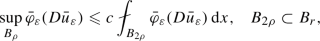for some $$c>0$$ depending only on n, p, $$q_1$$, $$\bar{\nu }$$ and $$\bar{\Lambda }$$. Set

\begin{aligned}{}[a_{ij}(\xi )] {:=} D_\xi A_\varepsilon (\xi ) \quad \text {and} \quad [b_{ij}(\xi )] {:=} D_\xi A_\varepsilon (\xi )\frac{\varepsilon +|\xi |}{\varphi '(\varepsilon +|\xi |)}. \end{aligned}

Note that, by (4.1), $$b_{ij}(\xi )$$ satisfies

\begin{aligned} \bar{\nu }|\tilde{\xi }|^2 \leqslant \sum _{i,j=1}^{n}b_{ij}(\xi ) \tilde{\xi }_i \tilde{\xi }_j \quad \text {and} \quad \big |[b_{ij}(\xi )]\big | \leqslant \bar{\Lambda }. \end{aligned}

Therefore, by following [28, Appendix A], in particular from the paragraph containing (A.7), with the above setting, we can obtain the desired regularity estimates for $$u_\varepsilon$$, where relevant constants are independent of $$\varepsilon$$.

Then using (4.2) and the uniform monotonicity (3.3) with $$A={\bar{A}}_{\varepsilon }$$ and $$\varphi ={\bar{\varphi }}_\varepsilon$$, we see that

\begin{aligned} {\bar{u}}_\varepsilon \ \ \longrightarrow \ \ {\bar{u}}\quad \text {in}\ \ W^{1,{\bar{\varphi }}}(B_r) \ \ \text {as}\ \ \varepsilon \rightarrow 0. \end{aligned}

Note that the standard Minty-Browder technique for a monotonicity operator, that is, an operator A which satisfies inequality (3.3) with right hand side 0, implies only weak convergence, but due to the uniform monotonicity we have the strong convergence. In particular, $$D{\bar{u}}_\varepsilon (x)$$ converges, up to a subsequence, to $$D{\bar{u}}(x)$$ almost everywhere in $$B_r$$.

The next result is a Calderón–Zygmund type estimate in the generalized Orlicz space for non-zero boundary data. Since $$\theta$$ is superlinear, this lemma allows us to transfer regularity from u to $${\bar{u}}$$.

### Lemma 4.5

Let $${\bar{A}}:\mathbb {R}^n\rightarrow \mathbb {R}^n$$ satisfy Assumption 1.1 with $$A(x,\xi )\equiv {\bar{A}}(\xi )$$ and constants $$L\geqslant 1$$ and $$1<p\leqslant q$$, and $${\bar{\varphi }}\in {\Phi _{\mathrm {c}}}$$ be its growth function. Suppose $$\theta \in {\Phi _{\mathrm {w}}}(B_r)$$ satisfies (A0), (aInc)$$_{p_\theta }$$ and (aDec)$$_{q_\theta }$$ with constants $$L_\theta \geqslant 1$$ and $$1<p_\theta \leqslant q_\theta$$ and (A1) with constant $$\bar{L}_K>0$$, and $$u\in W^{1,{\bar{\varphi }}}(B_r)$$ satisfies $$\int _{B_r}\theta (x,{\bar{\varphi }}(|Du|))\,\mathrm{d}x\leqslant \kappa$$ for some $$\kappa >0$$. If $${\bar{u}}\in u+W^{1,{\bar{\varphi }}}_0(B_r)$$ is a weak solution to ($$\mathrm {div}{\bar{A}}$$), then

\begin{aligned} \Vert {\bar{\varphi }}(|D{\bar{u}}|)\Vert _{L^\theta (B_r)} \leqslant c\, \Vert {\bar{\varphi }}(|Du|)\Vert _{L^\theta (B_r)} \end{aligned}

and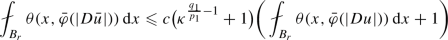for $$c=c(n,p,q,L, p_\theta ,q_\theta ,L_\theta ,\bar{L}_1)>0$$.

### Outline of proof

The lemma is a general version of [28, Lemma 4.15] and the proof is exactly the same if we have the general version of [28, Theorem B.1]. We note that the main tools used in the proof are weighted $$L^p$$ estimates, extrapolation in the generalized Orlicz spaces and scaling. In particular, [28, Theorem B.1] is the global weighted $$L^p$$ estimates for the gradient of weak solution to equation ($$\mathrm {div}{\bar{A}}$$) with $${\bar{A}}(\xi )={\bar{\varphi }}'(|\xi |)\frac{\xi }{|\xi |}$$.

The general version of [28, Theorem B.1] can be proved in a similar way. The major difference is establishing the estimate (see the next paragraph) concerned with the boundary Lipschitz regularity for the following approximate equation:

\begin{aligned} \mathrm {div}{\bar{A}}(D{\bar{v}})=0 \quad \text {in }\ B_{\rho }^+, \qquad {\bar{v}}=0 \quad \text {on }\ B_{\rho }(0)\cap \{x_n=0\}, \end{aligned}

where $$B_{\rho }^+=B_{\rho }(0)\cap \{x_n>0\}$$. Other differences are minor modifications.

For a weak solution $${\bar{v}}\in W^{1,{\bar{\varphi }}}(B_\rho ^+)$$ to the above equation, one can prove that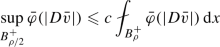for some $$c>0$$ depending only on n, p, $$q_1$$, $$\nu$$ and $$\Lambda$$. This corresponds to [28, (B.13)] in the Uhlenbeck case, which was obtained from the interior counterpart by applying the reflection argument with the odd extension, see the last paragraph in . However, in the general case the reflection argument does not work, and we use a so-called barrier argument. For the proof of the above Lipschitz estimate we refer to [11, Theorem 4.1], see also [10, Theorem 2.2]. Note that Cho  proved a Calderón-Zymund type estimate with $$\theta (x,t)\equiv \theta (t)$$ for nonhomogeneous equations with the zero boundary condition.

## 5 Approximation

In this section we construct approximations for A in ($$\mathrm {div}A$$) and F in ($$\min F$$). We start by recalling the simpler construction for $$\varphi \in C^{1}([0,\infty ))$$ with $$\varphi '$$ satisfying (A0), (Inc)$$_{p-1}$$ and (Dec)$${_{q_1}-1}$$ for some $$1<p\leqslant q_1$$. Let $$B_r=B_r(x_0)\subset \Omega$$ and $$0<t_1<1<t_2$$. We define

\begin{aligned} {\bar{\varphi }}(t){:=}\int _0^t {\bar{\varphi }}'(s)\,\mathrm{d}s \quad \text {with}\quad {\bar{\varphi }}'(t){:=} {\left\{ \begin{array}{ll} \frac{a_1}{t_1^{p-1}}t^{p-1}&{}\text {if} \ \ 0\leqslant t\leqslant t_1,\\ \varphi '(x_0,t)&{}\text {if}\ \ t_1\leqslant t\leqslant t_2,\\ \frac{a_2}{t_2^{p-1}}t^{p-1}&{}\text {if} \ \ t_2\leqslant t<\infty , \end{array}\right. } \end{aligned}
(5.1)

where $$a_1{:=}\varphi '(x_0, t_1)$$ and $$a_2 {:=} \varphi '(x_0, t_2)$$. Note that the relationship between $$\varphi$$ and $${\bar{\varphi }}$$ is exactly the same as in [28, Sect. 5]. From there, we have the following result.

### Proposition 5.1

Let $$\varphi$$ and $${\bar{\varphi }}$$ be from (5.1) and $$\tilde{L}\geqslant 1$$. Suppose that

\begin{aligned} \varphi _{B_r}^+(t)\leqslant \tilde{L}\varphi _{B_r}^-(t) \quad \text {for all }\ t\in [t_1,t_2]. \end{aligned}

Then,

1. (1)

$${\bar{\varphi }}\in C^1([0,\infty ))$$ with $${\bar{\varphi }}'$$ satisfying (Inc)$$_{p-1}$$ and (Dec)$${_{q_1}-1}$$.

2. (2)

$${\bar{\varphi }}(t)\leqslant \varphi (x_0,t)$$ and $$\tilde{L}^{-1} \varphi (x,t) \leqslant {\bar{\varphi }}(t)$$ for all $$(x,t)\in B_r\times [t_1,t_2]$$.

3. (3)

$${\bar{\varphi }}(t)\leqslant \frac{q_1}{p}\tilde{L} \varphi (x,t)$$ for all $$(x,t)\in B_r\times [t_1,\infty )$$.

4. (4)

$$\theta _0(x,t){:=}\varphi (x,{\bar{\varphi }}^{-1}(t))$$ satisfies (A0), (aInc)$$_{1}$$ and (aDec)$$_{q_1/p}$$ with constants L depending only on the structure constants of $$\varphi$$.

Note that the inequality assumed in the above proposition will be linked to the one in Proposition 3.6(2) and 3.7(2). In the Uhlenbeck case $$F(x,\xi )=\varphi (x,|\xi |)$$ a suitably mollified version of $${\bar{\varphi }}$$ can be used as the approximation of $$\varphi$$ . However, as far as we can tell, a similar approach to (5.1) does not work in the general case, so we introduce smooth transitions around $$t_1$$ and $$t_2$$ with transition functions $$\eta _i$$. We deal with A and F in separate subsections.

### 5.1 Approximation for weak solutions

Let $$A:\Omega \times {\mathbb {R}^n} \rightarrow {\mathbb {R}^n}$$ satisfy Assumption 1.1, and $$\varphi \in {\Phi _{\mathrm {c}}}(\Omega )$$ be its growth function. Recall that $$\varphi '$$ satisfies (A0), (Inc)$$_{p-1}$$ and (Dec)$${_{q_1}-1}$$. In this subsection, we construct an autonomous nonlinearity $${\bar{A}}:{\mathbb {R}^n} \rightarrow {\mathbb {R}^n}$$ with growth function $${\bar{\varphi }}$$ given by (5.1), such that $${\bar{A}}$$ and $${\bar{\varphi }}$$ are comparable with A and $$\varphi$$, respectively, in a suitable sense.

Fix any small ball $$B_r=B_r(x_0)\Subset \Omega$$ satisfying $$|B_r|\leqslant 1$$. For $$t_1\in (0,\frac{1}{2}]$$ and $$t_2 \geqslant 2$$ that will be chosen later in the next section, $$a_1$$ and $$a_2$$ from the definition of $${\bar{\varphi }}$$, and constants $$q_1$$, $$\nu$$, $$\Lambda$$ given in Proposition 3.3 we define

\begin{aligned} {\bar{A}}(\xi ){: =} \underbrace{\frac{\nu }{8} \eta _1(|\xi |) \frac{a_1}{t_1^{p-1}} |\xi |^{p-2}\xi }_{=:{\bar{A}}_1(\xi )} + \underbrace{\eta _2(|\xi |) A(x_0,\xi )}_{=:{\bar{A}}_2(\xi )} + \underbrace{\bar{\Lambda }\eta _3(|\xi |) \frac{a_2}{t_2^{p-1}} |\xi |^{p-2}\xi }_{=:{\bar{A}}_3(\xi )}, \end{aligned}
(5.2)

where $$\bar{\Lambda }{:=}\frac{2^{q_1 -p+3}\Lambda }{\min \{p-1,1\}}$$, and $$\eta _i\in C^\infty ([0,\infty ))$$, $$i=1,2,3$$, satisfy

\begin{aligned}&\eta _1\equiv 1\ \text { in } \ [0,t_1), \quad \eta _1\equiv 0\ \text { in } \ [2t_1,\infty ) \quad \text {and}\quad - \tfrac{2}{t_1}\leqslant \eta _1' \leqslant 0,\\&\eta _2\equiv 1\ \text { in } \ [0,t_2), \quad \eta _2\equiv 0\ \text { in } \ [2t_2,\infty ) \quad \text {and}\quad -\tfrac{2}{t_2} \leqslant \eta _2'\leqslant 0,\\&\eta _3\equiv 0\ \text { in } \ [0,\tfrac{t_2}{2}), \quad \eta _3\equiv 1\ \text { in } \ [t_2,\infty ) \quad \text {and}\quad 0\leqslant \eta _3'\leqslant \tfrac{4}{t_2}. \end{aligned}

Clearly, $${\bar{A}}\in C({\mathbb {R}^n} ,{\mathbb {R}^n} )\cap C^{1}({\mathbb {R}^n} \setminus \{ 0\},{\mathbb {R}^n} )$$, and

\begin{aligned} {\bar{A}}(\xi )=A(x_0,\xi ) \quad \text {whenever }\ 2t_1\leqslant |\xi | \leqslant \tfrac{t_2}{2}. \end{aligned}

Possible functions $$\eta _i$$ are sketched in Fig. 1 to assist in following the proof.

### Lemma 5.2

If $$\varphi$$ is a growth function of A, and $${\bar{\varphi }}$$ and $${\bar{A}}$$ are their autonomous approximations as in (5.1) and (5.2), then $${\bar{\varphi }}$$ is a growth function of $${\bar{A}}$$.

### Proof

Let us start by calculating the derivative of $$\eta _i(|\xi |) |\xi |^{p-2}\xi$$ with $$i=1,3$$:

\begin{aligned} D_\xi (\eta _i(|\xi |) |\xi |^{p-2}\xi ) = \eta _i'(|\xi |) |\xi |^{p-3}\xi \otimes \xi + (p-2)\eta _i(|\xi |) |\xi |^{p-4}\xi \otimes \xi + \eta _i(|\xi |) |\xi |^{p-2}I_n. \end{aligned}
(5.3)

Here $$\otimes$$ denotes the tensor product $$(a_i)_i\otimes (b_i)_j = (a_ib_j)_{i,j}$$ and $$I_n$$ is the n-dimensional identity matrix.

We consider the first condition (3.1) of being a growth function with $${\bar{A}}$$ and $${\bar{\varphi }}$$ in place of A and $$\varphi$$. When $$|\xi |<2t_1$$, we use $$|\eta _1'(t)|\,t \leqslant 2\frac{t}{t_1}\leqslant 4$$ and $${\bar{\varphi }}'(t)\approx \frac{a_1}{t^{p-1}_1}t^{p-1}$$ by (Inc)$$_{p-1}$$ and (Dec)$${_{q_1}-1}$$ for $$t_1\leqslant t <2t_1$$ to conclude that

\begin{aligned} |{\bar{A}}_1(\xi )| + |\xi | |D_\xi {\bar{A}}_1(\xi )|&\leqslant \frac{\nu }{8} \frac{a_1}{t_1^{p-1}} \big [|\xi |^{p-1}+|\eta _1'(|\xi |)| |\xi |^{p}+(p-1) |\xi |^{p-1}\big ]\\&\lesssim \frac{a_1}{t^{p-1}_1}|\xi |^{p-1} \approx {\bar{\varphi }}'(|\xi |). \end{aligned}

Similarly, we conclude that $$|{\bar{A}}_3(\xi )|+ |\xi | |D_\xi {\bar{A}}_3(\xi )|\lesssim \frac{a_2}{t_2^{p-1}}|\xi |^{p-1}\approx {\bar{\varphi }}'(|\xi |)$$ when $$|\xi |\geqslant \frac{1}{2}t_2$$. Finally, it follows by (3.1) of A and $$\varphi '(x_0,t)\approx \varphi '(x_0,t_2)$$ when $$t_1\leqslant t \leqslant 2t_2$$ that $$|{\bar{A}}_2(\xi )|+ |\xi | |D_\xi {\bar{A}}_2(\xi )|\lesssim {\bar{\varphi }}'(|\xi |)$$. Therefore, we have established (3.1) of $${\bar{A}}$$.

For the ellipticity condition (3.2), we consider four cases: $$0<|\xi |\leqslant t_1$$, $$t_1<|\xi |\leqslant 2 t_1$$, $$t_2/2<|\xi |\leqslant t_2$$ and $$t_2<|\xi |\leqslant 2 t_2$$. The strategy is to get the ellipticity condition by the same condition of $${\bar{A}}_1$$ and $${\bar{A}}_2$$ for first and third cases, respectively. In the second and fourth cases one term of $$D_\xi {\bar{A}}$$ is non-negative and the other is non-positive (since $$\eta _1'$$ or $$\eta _2'$$ is non-positive), so we have to show that the non-positive term can be absorbed in the non-negative one based on more precise estimates.

Note that the other cases, $$2t_1<|\xi |\leqslant t_2/2$$ and $$|\xi |>2t_2$$, are clear, since in these intervals only $${\bar{A}}_2$$ and $${\bar{A}}_3$$, respectively, influence $${\bar{A}}$$.

In this interval, $$\eta _1\equiv \eta _2\equiv 1$$ and $$\eta _3\equiv 0$$. By the calculation (5.3) and the ellipticity of $$A(x_0,\xi )$$,

\begin{aligned}\begin{aligned} D_\xi {\bar{A}}(\xi ) \tilde{\xi }\cdot \tilde{\xi }&= D_\xi {\bar{A}}_1(\xi ) \tilde{\xi }\cdot \tilde{\xi }+ \underbrace{D_\xi A(x_0, \xi ) \tilde{\xi }\cdot \tilde{\xi }}_{\geqslant 0}\\&\geqslant \frac{\nu }{8} \frac{a_1}{t_1^{p-1}} [(p-2) |\xi |^{p-4}(\xi \cdot \tilde{\xi })^2 + |\xi |^{p-2}|\tilde{\xi }|^2] \\&\geqslant \frac{\nu }{8} \min \{p-1,1\} \frac{a_1}{t_1^{p-1}} |\xi |^{p-2}|\tilde{\xi }|^2 = \frac{\nu }{8} \min \{p-1,1\} \frac{{\bar{\varphi }}'(|\xi |)}{|\xi |} |\tilde{\xi }|^2. \end{aligned}\end{aligned}

In this interval, $$-\frac{4}{|\xi |} \leqslant -\frac{2}{t_1} \leqslant \eta _1' \leqslant 0$$, $$\eta _2\equiv 1$$ and $$\eta _3\equiv 0$$. Therefore by the ellipticity of $$|\xi |^{p-2}\xi$$ and $$A(x_0,\xi )$$ and (Inc)$$_{p-1}$$ of $$\varphi '$$,

\begin{aligned}\begin{aligned} D_\xi {\bar{A}}(\xi ) \tilde{\xi }\cdot \tilde{\xi }&= \frac{\nu }{8} \eta '(|\xi |) \frac{a_1}{t_1^{p-1}} |\xi |^{p-3} (\xi \cdot \tilde{\xi })^2 +\underbrace{\frac{\nu }{8} \eta _1(|\xi |)\frac{a_1}{t_1^{p-1}}D_\xi (|\xi |^{p-2}\xi )\tilde{\xi }\cdot \tilde{\xi }}_{\geqslant 0}\\ {}&\qquad + D_\xi A(x_0,\xi ) \tilde{\xi }\cdot \tilde{\xi }\\ {}&\geqslant - \frac{\nu }{8} \frac{4}{|\xi |} \frac{a_1}{t_1^{p-1}} |\xi |^{p-1} |\tilde{\xi }|^2 + \nu \frac{\varphi '(x_0,|\xi |)}{|\xi |}|\tilde{\xi }|^2 \\ {}&\geqslant - \frac{\nu }{2} \frac{\varphi '(x_0,|\xi |)}{|\xi |}|\tilde{\xi }|^2 + \nu \frac{\varphi '(x_0,|\xi |)}{|\xi |}|\tilde{\xi }|^2 = \frac{\nu }{2} \frac{{\bar{\varphi }}'(|\xi |)}{|\xi |} |\tilde{\xi }|^2. \end{aligned}\end{aligned}

In this interval, $$\eta '_3,\eta _3\geqslant 0$$, $$\eta _2\equiv 1$$ and $$\eta _1\equiv 0$$. Hence

\begin{aligned}\begin{aligned} D_\xi {\bar{A}}(\xi ) \tilde{\xi }\cdot \tilde{\xi }&= D_\xi A(x_0,\xi ) \tilde{\xi }\cdot \tilde{\xi }+\underbrace{\bar{\Lambda }\eta _3'(|\xi |) \frac{a_2}{t_2^{p-1}} |\xi |^{p-3} (\xi \cdot \tilde{\xi })^2}_{\geqslant 0} \\ {}&\qquad +\underbrace{\bar{\Lambda }\eta _3(|\xi |) \frac{a_2}{t_2^{p-1}} \partial (|\xi |^{p-2}\xi ) \tilde{\xi }\cdot \tilde{\xi }}_{\geqslant 0}\\ {}&\geqslant D_\xi A(x_0,\xi ) \tilde{\xi }\cdot \tilde{\xi }\geqslant \nu \frac{\varphi '(x_0,|\xi |)}{|\xi |} |\tilde{\xi }|^2 =\nu \frac{{\bar{\varphi }}'(|\xi |)}{|\xi |} |\tilde{\xi }|^2. \end{aligned}\end{aligned}

In this interval, $$-\frac{4}{|\xi |} \leqslant -\frac{2}{t_2} \leqslant \eta _2'\leqslant 0$$, $$\eta _3\equiv 1$$ and $$\eta _1\equiv 0$$. Therefore

\begin{aligned}\begin{aligned} D_\xi {\bar{A}}(\xi ) \tilde{\xi }\cdot \tilde{\xi }&= \frac{\eta _2'(|\xi |)}{|\xi |} (\xi \otimes A(x_0,\xi ))\tilde{\xi }\cdot \tilde{\xi }+ \underbrace{\eta _2(|\xi |)D_\xi A(x_0,\xi ) \tilde{\xi }\cdot \tilde{\xi }}_{\geqslant 0} \\ {}&\qquad + \bar{\Lambda }\frac{a_2}{t_2^{p-1}} D_\xi (|\xi |^{p-2}\xi ) \tilde{\xi }\cdot \tilde{\xi }\\ {}&\geqslant - 4\Lambda \frac{\varphi '(x_0,|\xi |)}{|\xi |} |\tilde{\xi }|^2 + \bar{\Lambda }\min \{p-1,1\} \frac{a_2}{t_2^{p-1}} |\xi |^{p-2}|\tilde{\xi }|^2. \end{aligned} \end{aligned}

For the first term, we use (Inc)$$_{p-1}$$ and (Dec)$${_{q_1}-1}$$ of $$\varphi '$$ to conclude that

\begin{aligned} \frac{\varphi '(x_0,|\xi |)}{|\xi |^{p-1}} \leqslant \frac{\varphi '(x_0,2t_2)}{(2t_2)^{p-1}} \leqslant 2^{q_1-p}\frac{\varphi '(x_0,t_2)}{t_2^{p-1}} = 2^{q_1-p}\frac{a_2}{t_2^{p-1}}. \end{aligned}

Recalling the definition of $$\bar{\Lambda }$$, we complete the proof of (3.2) of $${\bar{A}}$$ with the observation

\begin{aligned} D_\xi {\bar{A}}(\xi ) \tilde{\xi }\cdot \tilde{\xi }\geqslant 2^{q_1-p+2}\Lambda \frac{a_2}{t_2^{p-1}} |\xi |^{p-2}|\tilde{\xi }|^2= 2^{q_1-p+2}\Lambda \frac{{\bar{\varphi }}'(|\xi |)}{|\xi |}|\tilde{\xi }|^2 . \end{aligned}

$$\square$$

### 5.2 Approximation for minimizers

Let $$F:\Omega \times {\mathbb {R}^n} \rightarrow [0,\infty )$$ satisfy Assumption 1.5 with constants $$L\geqslant 1$$ and $$1<p<q$$, and $$\varphi \in {\Phi _{\mathrm {c}}}(\Omega )$$ be its growth function. We construct an autonomous function $${\bar{F}}:{\mathbb {R}^n} \rightarrow [0,\infty )$$ with growth function $${\bar{\varphi }}$$ given in (5.1). The construction is similar to, yet more delicate than, that of the previous subsection. The added difficulty comes from the fact that we need to differentiate F twice, which makes controlling the approximation more challenging.

Fix any small ball $$B_r=B_r(x_0)\Subset \Omega$$ with $$r\in (0,1)$$ satisfying $$|B_r|\leqslant 1$$. For $$t_1\in (0,\frac{1}{2}]$$, $$t_2 \geqslant 2$$, $$\bar{\nu }\ll 1$$ and $$\bar{\Lambda }\gg 1$$ that will be chosen later, we define

\begin{aligned} {\bar{F}}(\xi ): = \underbrace{\bar{\nu }\eta _1(|\xi |) \frac{a_1}{t_1^{p-1}} |\xi |^{p}}_{=:{\bar{F}}_1(\xi )} + \underbrace{\eta _2(|\xi |) F(x_0,\xi ) }_{=:{\bar{F}}_2(\xi )} + \underbrace{\bar{\Lambda }\eta _3(|\xi |) \frac{a_2}{t_2^{p-1}} |\xi |^p}_{=:{\bar{F}}_3(\xi )}, \end{aligned}
(5.4)

where $$\eta _i\in C^\infty ([0,\infty ))$$, $$i=1,2,3$$, satisfy

\begin{aligned}&\eta _1\equiv 1\ \text { in } \ [0,t_1), \quad \eta _1\equiv 0\ \text { in } \ [2t_1,\infty ) ,\quad \eta _1' \leqslant 0 \quad \text {and}\quad |\eta _1'|t_1 +|\eta _1''|t_1^2 \leqslant 10,\\&\eta _2\equiv 1\ \text { in } \ [0,t_2), \quad \eta _1\equiv 0\ \text { in } \ [2t_2,\infty ) ,\quad \eta _2'\leqslant 0 \quad \text {and}\quad |\eta _2'|t_2 + |\eta _2''|t_2^2 \leqslant 10,\\&\eta _3(t){:=}\int _0^t \frac{h(s)}{s^2}\, \mathrm{d}s \end{aligned}

with $$h\in C^\infty ([0,\infty ))$$ increasing, equal to 0 on $$[0,\frac{1}{2} t_2]$$ and to $$t_2$$ on $$[\frac{3}{4} t_2, \infty ]$$, and $$\Vert h'\Vert _\infty \leqslant 10$$.

Observe that $$\eta _1$$ and $$\eta _2$$ are analogous to their namesakes in the previous subsection, but $$\eta _3$$ behaves somewhat differently. The reason is that if $$\eta _3$$ is forced to be constant from $$2t_2$$ onward as in the previous subsection, then it is not possible to control derivatives up to order 2 in an appropriate manner. We again note that

\begin{aligned} \eta _i(t) + t |\eta _i'(t)| + t^2 |\eta _i''(t)| \leqslant C \end{aligned}
(5.5)

for $$i=1, 2, 3$$ and all $$t\geqslant 0$$, and that

\begin{aligned} {\bar{F}}(\xi )=F(x_0,\xi ) \quad \text {whenever }\ 2t_1\leqslant |\xi | \leqslant \tfrac{t_2}{2}. \end{aligned}

### Lemma 5.3

If $$\varphi$$ is a growth function of F, and $${\bar{\varphi }}$$ and $${\bar{F}}$$ are their autonomous approximations as in (5.1) and (5.4), then $${\bar{\varphi }}$$ is a growth function of $${\bar{F}}$$.

### Proof

To check the first condition of the definition of growth function, (3.1) with $$A{:=}D_\xi {\bar{F}}$$ and $$\varphi {:=}{\bar{\varphi }}$$, we calculate the derivatives and use (5.5) as in Lemma 5.2; the only noteworthy feature is that we use (Inc)$$_{p-1}$$ to control $$F(x_0,\xi )$$ and its derivatives when $$t<t_1$$:

\begin{aligned} |D_\xi {\bar{F}}(\xi )| \leqslant p\bar{\nu }a_1 (\tfrac{|\xi |}{t_1})^{p-1}+ \Lambda \varphi '(x_0,|\xi |) \leqslant (p\bar{\nu }+\Lambda )a_1(\tfrac{|\xi |}{t_1})^{p-1} \approx {\bar{\varphi }}'(|\xi |) \end{aligned}

and

\begin{aligned} |\xi |\,|D_\xi ^2{\bar{F}}(\xi )|&\leqslant c(n,p)\bar{\nu }a_1 (\tfrac{|\xi |}{t_1})^{p-1} + \Lambda \varphi '(x_0,|\xi |) \\&\leqslant (c(n,p) \bar{\nu }+\Lambda ) a_1(\tfrac{|\xi |}{t_1})^{p-1} \approx {\bar{\varphi }}'(|\xi |). \end{aligned}

We move on to (3.2) and consider four cases: $$0<|\xi |\leqslant t_1$$, $$t_1<|\xi |\leqslant 2 t_1$$, $$t_2/2<|\xi |\leqslant t_2$$ and $$|\xi |>t_2$$. The remaining case $$2t_1<|\xi |\leqslant t_2/2$$ follows from the assumption that $$\varphi$$ is a growth function of F, since $$\varphi \equiv {\bar{\varphi }}$$ and $$F\equiv {\bar{F}}$$ in this set.

As in Lemma 5.2, we find that

\begin{aligned}\begin{aligned} D_\xi ^2{\bar{F}}(\xi ) \tilde{\xi }\cdot \tilde{\xi }&= \bar{\nu }\frac{a_1}{t_1^{p-1}}D_\xi (|\xi |^{p-2}\xi )\tilde{\xi }\cdot \tilde{\xi }+ \underbrace{D_\xi ^2F(x_0,\xi ) \tilde{\xi }\cdot \tilde{\xi }}_{\geqslant 0} \\&\geqslant \bar{\nu }\min \{p-1,1\} \frac{a_1}{t_1^{p-1}} |\xi |^{p-2}|\tilde{\xi }|^2 = \bar{\nu }\min \{p-1,1\}\frac{{\bar{\varphi }}'(|\xi |)}{|\xi |} |\tilde{\xi }|^2. \end{aligned}\end{aligned}

We calculate that

\begin{aligned}\begin{aligned} D_\xi {\bar{F}}(\xi )&= \bar{\nu }\big ( \eta _1'(|\xi |)|\xi |+p\eta _1(|\xi |)\big ) \frac{a_1}{t_1^{p-1}} |\xi |^{p-2}\xi + D_\xi F(x_0,\xi ) \end{aligned}\end{aligned}

and

\begin{aligned}\begin{aligned} D_\xi ^2{\bar{F}}(\xi )&= \bar{\nu }\big [ \eta _1''(|\xi |)|\xi |^2+ (1+p)\eta _1'(|\xi |)|\xi |\big ] \frac{a_1}{t_1^{p-1}} |\xi |^{p-4} \xi \otimes \xi \\&\qquad + \bar{\nu }\big [ \eta _1'(|\xi |)|\xi |+p\eta _1(|\xi |)\big ] \frac{a_1}{t_1^{p-1}} D_\xi (|\xi |^{p-2}\xi ) + D_\xi ^2 F(x_0,\xi ). \end{aligned}\end{aligned}

By (5.5), the coefficients in the square brackets are bounded from below by a negative constant. By taking $$\bar{\nu }$$ sufficiently small and using (Inc)$$_{p-1}$$ and (Dec)$$_{q-1}$$ of $${\bar{\varphi }}'$$, we have that

\begin{aligned}\begin{aligned} D_\xi ^2{\bar{F}}(\xi ) \tilde{\xi }\cdot \tilde{\xi }&\geqslant - \bar{\nu }c \frac{a_1}{t_1^{p-1}} |\xi |^{p-2} |\tilde{\xi }|^2 + \nu \frac{\varphi '(x_0,|\xi |)}{|\xi |}|\tilde{\xi }|^2 \geqslant \frac{\nu }{2} \frac{\varphi '(x_0, |\xi |)}{|\xi |}|\tilde{\xi }|^2 \\&\approx \frac{\nu }{2} \frac{{\bar{\varphi }}'(|\xi |)}{|\xi |}|\tilde{\xi }|^2 . \end{aligned}\end{aligned}

We observe that $$\eta _3'(t)=\frac{h(t)}{t^2}$$. Since $$h(t)=\eta _3'(t)t^2$$ is increasing and differentiable, we conclude that $$(\eta _3'(t)t^2)'=\eta _3''(t)t^2 + 2t\eta _3'(t)\geqslant 0$$. Also $$p\eta _3(t)+ \eta _3'(t)t\geqslant 0$$ since both terms are non-negative. We calculate $$D_\xi {\bar{F}}_3(\xi )= \bar{\Lambda }\big ( p\eta _3(|\xi |)+ \eta _3'(|\xi |) |\xi |\big ) \frac{a_2}{t_2^{p-1}} |\xi |^{p-2}\xi$$ and

\begin{aligned} D_\xi ^2{\bar{F}}_3(\xi ) \tilde{\xi }\cdot \tilde{\xi }&= \bar{\Lambda }\big ( (p+1)\eta _3'(|\xi |)|\xi |+ \eta _3''(|\xi |)|\xi |^2 \big )\frac{a_2}{t_2^{p-1}} |\xi |^{p-4} (\xi \cdot \tilde{\xi })^2\\&\qquad + \bar{\Lambda }\big ( p\eta _3(|\xi |)+ \eta _3'(|\xi |)|\xi |\big ) \frac{a_2}{t_2^{p-1}}D_\xi (|\xi |^{p-2}\xi ) \tilde{\xi }\cdot \tilde{\xi }\geqslant 0. \end{aligned}

Therefore, the ellipticity of $${\bar{F}}$$ follows from the ellipticity of $${\bar{F}}_2(\xi )=A(x_0,\xi )$$.

As in the previous case, we conclude that

\begin{aligned} D_\xi ^2{\bar{F}}_3(\xi )\tilde{\xi }\cdot \tilde{\xi }&\geqslant \bar{\Lambda }p\eta _3(|\xi |) \frac{a_2}{t_2^{p-1}} D_\xi (|\xi |^{p-2}\xi ) \tilde{\xi }\cdot \tilde{\xi }\\&\geqslant \tfrac{\bar{\Lambda }p}{3} \min \{1,p-1\} \frac{a_2}{t_2^{p-1}}|\xi |^{p-2}|\tilde{\xi }|^2, \end{aligned}

where we used that $$\eta _3'\geqslant 0$$ and

\begin{aligned} \eta _3(|\xi |) \geqslant \eta _3(t_2) = \int _0^{t_2} \frac{h(s)}{s^2} \, \mathrm{d}s \geqslant t_2\int _{\frac{3}{4} t_2}^{t_2} \frac{1}{s^2} \, \mathrm{d}s = \frac{1}{3}. \end{aligned}

Hence the ellipticity of $${\bar{F}}={\bar{F}}_3$$ when $$|\xi |>2t_2$$ follows.

Finally, we suppose that $$t_2<|\xi |\leqslant 2 t_2$$. We calculate $$D_\xi {\bar{F}}_2(\xi )= \frac{\eta _2'(|\xi |)}{|\xi |} F(x_0,\xi ) \xi + \eta _2(|\xi |)D_\xi F(x_0,\xi )$$ and

\begin{aligned} \begin{aligned} D_\xi ^2{\bar{F}}_2(\xi ) =&\frac{\eta _2'(|\xi |)}{|\xi |} F(x_0,\xi ) I_n + 2\frac{\eta _2'(|\xi |)}{|\xi |} D_\xi F(x_0,\xi ) \otimes \xi \\&\quad +\frac{\eta _2''(|\xi |)|\xi |-\eta _2'(|\xi |)}{|\xi |^3} F(x_0,\xi ) \xi \otimes \xi \\&\quad + \eta _2(|\xi |)D_\xi ^2F(x_0,\xi ) . \end{aligned} \end{aligned}

Since $$|\eta _2'|t_2+|\eta _2''|t_2^2 \leqslant 10$$ and $$|\xi |\approx t_2$$, by choosing $$\bar{\Lambda }$$ sufficiently large for the second estimate, we obtain that

\begin{aligned}\begin{aligned} D_\xi ^2{\bar{F}}(\xi ) \tilde{\xi }\cdot \tilde{\xi }&\geqslant - c \frac{\varphi '(x_0,|\xi |)}{|\xi |} |\tilde{\xi }|^2 + \tfrac{\bar{\Lambda }p}{3} \min \{p-1,1\} \frac{a_2}{t_2^{p-1}}|\xi |^{p-2} |\tilde{\xi }|^2\\&\gtrsim \frac{{\bar{\varphi }}'(|\xi |)}{|\xi |^{p-1}} |\tilde{\xi }|^2; \end{aligned}\end{aligned}

here we also used (Inc)$$_{p-1}$$ and (Dec)$${_{q_1}-1}$$ of $$\varphi '$$ to conclude that

\begin{aligned} \frac{\varphi '(x_0,|\xi |)}{|\xi |^{p-1}} \leqslant \frac{\varphi '(x_0,2t_2)}{(2t_2)^{p-1}} \leqslant 2^{q_1-p}\frac{\varphi '(x_0,t_2)}{t_2^{p-1}} = 2^{q_1-p}\frac{{\bar{\varphi }}'(|\xi |)}{|\xi |^{p-1}}. \end{aligned}

Thus we obtain the second condition of being a growth function, (3.2).

## 6 Regularity of Weak Solutions and Minimizers

In this section we prove the main theorems stated in the introduction. We consider either $$A:\Omega \times {\mathbb {R}^n} \rightarrow {\mathbb {R}^n}$$ satisfying Assumption 1.1 with $$A^{(-1)}$$ satisfying (wVA1) or $$F:\Omega \times {\mathbb {R}^n} \rightarrow \mathbb {R}$$ satisfying Assumption 1.5 and (wVA1). Note that the parameters p, q and L are from Assumption 1.1 or 1.5, and the parameter $$\bar{L}$$ is given by $$\bar{L}_K$$ in (wVA1) with

\begin{aligned} K=2c_1 \ \ \text {in the equation case}\ \ \text {or}\ \ K=2c_2 \ \ \text {in the functional case,} \end{aligned}
(6.1)

where $$c_1$$ and $$c_2$$ are from Propositions 3.6 and 3.7. Let $$\varphi$$ be a growth function of A or F. Note that by Proposition 3.6 or  3.7, $$A^{(-1)}$$ or F satisfies (A1) when $$K=1$$ with $$\bar{L}_1$$ depending only on n, p, q, L and $$\bar{L}$$, hence so does $$\varphi$$.

Let $$u\in W^{1,\varphi }_{\mathrm {loc}}(\Omega )$$ be a local weak solution to ($$\mathrm {div}A$$) or a local minimizer of ($$\min F$$). Then, by Theorem 4.1 along with Remark 4.2, we have $$\varphi (\cdot ,|Du|)\in L^{1+\sigma }_{\mathrm {loc}}(\Omega )$$ for some $$\sigma =\sigma (n,p,q,L,\bar{L}) \in (0,1)$$. With this $$\sigma$$, we fix the parameters $$\varepsilon$$ and $$\omega =\omega _\varepsilon$$ from (wVA1) by

\begin{aligned} \varepsilon {:=} \frac{\sigma }{2(2+\sigma )}<\frac{1}{6}, \end{aligned}
(6.2)

We also fix $$\Omega '\Subset \Omega$$, and consider any $$B_{2r}\subset \Omega '$$ with $$r\in (0,\frac{1}{2})$$ satisfying

\begin{aligned} \omega (r)\leqslant \frac{1}{2^{q_1}L}, \ \ |B_{2r}| \leqslant \max \left\{ 2^{p}L, 2^{\frac{1}{1-\varepsilon }}, 2^{\frac{2(1+\sigma )}{\sigma }} \Vert \varphi (\cdot ,|Du|)^{1+\sigma }\Vert _{L^1(\Omega ')}^{\frac{2+\sigma }{\sigma }}\right\} ^{-1}. \end{aligned}

Note that using Hölder’s inequality we have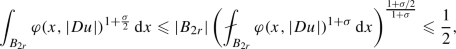(6.3)

so that

\begin{aligned} \int _{B_{2r}}\varphi (x,|Du|)\,\mathrm{d}x\leqslant \int _{B_{2r}}\varphi (x,|Du|)^{1+\frac{\sigma }{2}}\,\mathrm{d}x+ |B_{2r}|\leqslant \frac{1}{2}+\frac{1}{2} = 1. \end{aligned}
(6.4)

Note that this allows us to take advantage of the higher integrability estimate in Theorem 4.1. Next we set

\begin{aligned} t_1{:=} (\varphi ^-_{B_r})^{-1}(\omega (r)) \leqslant \frac{1}{2}\quad \text {and}\quad t_2{:=}(\varphi ^-_{B_r})^{-1}(|B_{r}|^{-1})\geqslant 2. \end{aligned}

With these $$t_1$$ and $$t_2$$, we construct $${\bar{A}}$$, $${\bar{F}}$$ and $${\bar{\varphi }}$$ as described in Sect. 5.

### 6.1 Regularity for weak solutions

We first prove Theorem 1.2. We assume $$A:\Omega \times {\mathbb {R}^n} \rightarrow {\mathbb {R}^n}$$ satisfies Assumptions 1.1 and $$A^{(-1)}$$ satisfies (wVA1), and consider a local weak solution $$u\in W^{1,\varphi }_{\mathrm {loc}}(\Omega )$$ to ($$\mathrm {div}A$$). By Proposition 3.6(1),

\begin{aligned} |A(x,\xi )-A(y,\xi )|\lesssim \omega (r)^\frac{1}{p'}\big ((\varphi ')^-_{B_r}(|\xi |)+1\big ), \end{aligned}
(6.5)

for all $$\xi \in \mathbb {R}^n$$ satisfying $$\varphi _{B_r}^-(|\xi |)\in \left[ 0,|B_r|^{-1+\varepsilon }\right]$$. Moreover, by Proposition 3.6(2), the condition of Proposition 5.1 holds with $$\tilde{L}=\tilde{L}(n,p,q,L,\bar{L})$$.

Recall that $${\bar{\varphi }}$$ and $${\bar{A}}$$ were constructed in (5.1) and (5.2). Let $${\bar{u}}\in u+ W^{1,{\bar{\varphi }}}_0(B_r)$$ be a weak solution to ($$\mathrm {div}{\bar{A}}$$). By Proposition 5.1(3), $$u\in W^{1,{\bar{\varphi }}}(B_r)$$, so it is a suitable boundary value. The following lemma is a generalization of [28, Lemma 5.15] to the non-Uhlenbeck case. Note that we use only the (A1) condition of $$A^{(-1)}$$ in the proof.

### Lemma 6.1

Let A, $$\varphi$$, u, $$\bar{L}$$, $$\sigma$$, $$B_{2r}$$ and $${\bar{u}}$$ be as above. Then

The implicit constants depend only on n, p, q, L and $$\bar{L}$$.

### Proof

By Hölder’s inequality and higher integrability in Theorem 4.1, we have that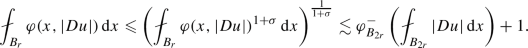From (6.4) we obtain that. Hence (1) follows by Proposition 5.1(2).

By Proposition 5.1(4), we see that $$\theta (x,t){:=}\varphi (x,{\bar{\varphi }}^{-1}(t))^{1+\sigma /2}$$ satisfies (A0), (aInc)$$_{1+\sigma /2}$$ and (aDec)$$_{(1+\sigma /2)q_1/p}$$. Moreover, (A1) of $$\varphi$$ implies (A1) of $$\theta$$. Therefore, in view of Lemma 4.5 with (6.3) we have (2).

Finally, by Jensen’s inequality, the standard energy estimate for ($$\mathrm {div}{\bar{A}}$$), i.e. the estimate in Lemma 4.5 with $$\theta (x, t)\equiv t$$, and (1), we have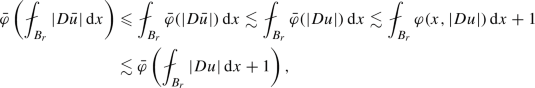which implies (3), since $${\bar{\varphi }}$$ is strictly increasing.

We next estimate the difference of the gradient between u and $${\bar{u}}$$ in the $$L^1$$-sense. This generalizes Lemma 6.2 and Corollary 6.3 of  to the non-Uhlenbeck case.

### Lemma 6.2

Let A, $$\varphi$$, u, $$\bar{L}$$, $$\varepsilon$$, $$\omega$$, $$B_{2r}$$, $${\bar{\varphi }}$$, $${\bar{A}}$$ and $${\bar{u}}$$ be as above. Then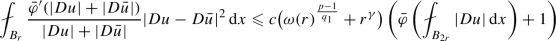andfor some $$c\geqslant 1$$ and $$\gamma \in (0,1)$$ depending only on n, p, q, L and $$\bar{L}$$.

### Proof

Using the monotonicity of $${\bar{A}}$$ (3.3) and taking $$u-{\bar{u}}\in W^{1,\varphi }_0(B_r)\subset W^{1,{\bar{\varphi }}}_0(B_r)$$ as a test function in the weak forms of ($$\mathrm {div}A$$) and ($$\mathrm {div}{\bar{A}}$$), we find that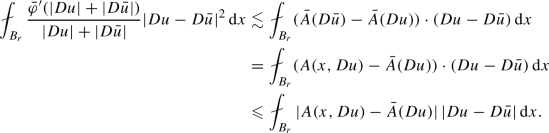We split $$B_r$$ into two regions defined by

\begin{aligned} \begin{aligned} E_1{:=}B_r \cap \Big \{ \varphi ^-_{B_r}(|Du|)\leqslant \frac{|B_{r}|^{-1+\varepsilon }}{2^{p}}\Big \} \quad \text {and}\quad E_2{:=}B_r \cap \Big \{\frac{|B_{r}|^{-1+\varepsilon }}{2^p }<\varphi ^-_{B_r}(|Du|)\Big \}. \end{aligned} \end{aligned}

In the set $$E_1$$, we have $$|Du|\leqslant \frac{t_2}{2}$$. If also $$2t_1 \leqslant |Du|$$, then it follows from $${\bar{A}}(\xi )=A(x_0, \xi )$$ and (6.5) that

\begin{aligned} |A(x,Du)- {\bar{A}}(Du)|=|A(x,Du)- A(x_0,Du)| \lesssim \omega (r)^{1-\frac{1}{p}} ((\varphi ')^-(|Du|)+1). \end{aligned}

If, on the other hand, $$|Du|\leqslant 2t_1$$, then (Dec)$$_{q_1}$$ and (A0) of $$\varphi$$ imply that $$|Du|\lesssim \omega (r)^\frac{1}{q_1}$$. Therefore by (Inc)$$_{p-1}$$ and (A0) of $$\varphi '$$ and $${\bar{\varphi }}'$$ and the growth function property (3.1) of A and $${\bar{A}}$$, we see that $$|A(x,Du)- {\bar{A}}(Du)|\lesssim \omega (r)^\frac{p-1}{q_1}$$. Applying Young’s inequality with $$\varphi ^-_{B_r}$$ and $$(\varphi ^-_{B_r})^*$$ and $$\varphi ^*(x,\varphi '(x,t))\leqslant \varphi (x,t)$$ as well as $$t\lesssim \varphi (x,t)+1$$, we find that

\begin{aligned}\begin{aligned} |A(x,Du)-{\bar{A}}(Du)|\,|Du-D{\bar{u}}| \chi _{E_1}&\lesssim \omega (r)^\frac{p-1}{q_1} [(\varphi ')^-(|Du|)|Du-D{\bar{u}}| + |Du-D{\bar{u}}|]\\&\lesssim \omega (r)^\frac{p-1}{q_1} [\varphi (x,|Du|)+ \varphi (x,|D{\bar{u}}|)+1]. \end{aligned}\end{aligned}

Next we integrate this inequality over $$B_r$$ and use Lemma 6.1(1) &(2):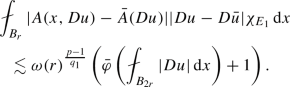In the set $$E_2$$, $$1\lesssim |B_{r}|^{1-\varepsilon }\varphi ^-_{B_r}(|Du|)$$. Then applying Young’s inequality and Proposition 5.1(3) we have

\begin{aligned}\begin{aligned} |A(x,Du)-{\bar{A}}(Du)|\,|Du-D{\bar{u}}| \chi _{E_2}&\lesssim \varphi '(x,|Du|)|Du-D{\bar{u}}| \lesssim \varphi (x,|Du|) + \varphi (x,|D{\bar{u}}|)\\&\lesssim \left[ |B_{2r}|^{1-\varepsilon }\varphi ^-_{B_r}(|Du|)\right] ^{\frac{\sigma }{2}} [\varphi (x,|Du|) + \varphi (x,|D{\bar{u}}|)]\\&\lesssim r^{\frac{n(1-\varepsilon )\sigma }{2}}\big ( \varphi (x,|Du|)^{1+\frac{\sigma }{2}}+\varphi (x,|D{\bar{u}}|)^{1+\frac{\sigma }{2}}\big ). \end{aligned}\end{aligned}

Integrating this inequality over $$B_r$$ and using Lemma 6.1(2) and the definition of $$\varepsilon$$ from (6.2), we find that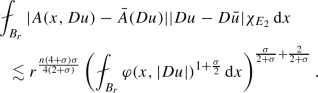On one hand, by Lemma 6.1(1), we have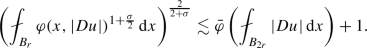On the other hand, by (6.3),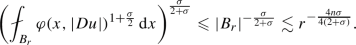Therefore, the previous three inequalities imply that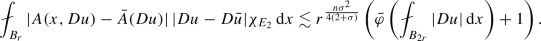Combining the results of this and the previous paragraph, we have the first claim of the lemma, with $$\gamma {:=}\frac{n\sigma ^2}{4(2+\sigma )}$$.

Next, set $$\omega _0(r){:=}\omega (r)^\frac{p-1}{q_1}+r^\gamma$$. Applying Proposition 2.4(3) with $$\kappa =\omega _0(r)^{\frac{1}{2}}$$, Proposition 5.1(3), Lemma 6.1(1) &(2) and the first part of the lemma, we find that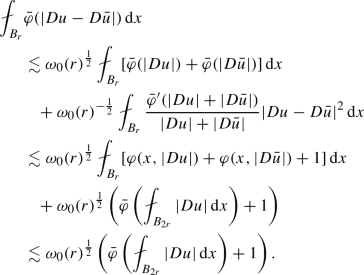Therefore, by Jensen’s inequality and (Dec)$$_{q_1}$$ of $${\bar{\varphi }}$$, we have that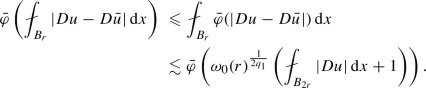Since $${\bar{\varphi }}$$ is strictly increasing, this implies the second claim of the lemma.

Now, the regularity result for weak solutions follows.

### Proof of Theorem 1.2

We can prove the theorem using Lemmas 4.4, 6.1 and 6.2 using a standard iteration argument. The proof is exactly the same as [28, Theorem 7.2], see also [1, 2], so we omit it.

### 6.2 Regularity for minimizers

In this subsection we prove Theorem 1.6. The method is almost the same as for Theorem 1.2 except for the comparison step. Hence we will take advantage of many parts from the previous subsection.

We assume $$F:\Omega \times {\mathbb {R}^n} \rightarrow \mathbb {R}$$ satisfies Assumption 1.5 and (wVA1), and consider a local minimizer $$u\in W^{1,\varphi }_{\mathrm {loc}}(\Omega )$$ of ($$\min F$$). Recall that the minimizer u is also a weak solution to ($$\mathrm {div}A$$) with $$A=D_\xi F$$, but (wVA1) of F does not imply (wVA1) of $$A{:=}D_\xi F$$. On the other hand, by Proposition 3.7(1) we have

\begin{aligned} F^+_{B_r}(\xi )-F^-_{B_r}(\xi ) \lesssim \omega (r)\big (\varphi ^-_{B_r}(|\xi |)+1\big ), \end{aligned}
(6.6)

for all $$\xi \in \mathbb {R}^n$$ satisfying $$\varphi _{B_r}^-(|\xi |)\in \left[ 0,|B_r|^{-1+\varepsilon }\right]$$. Moreover, by the Proposition 3.7(2), the condition of Proposition 5.1 holds with $$\tilde{L}=\tilde{L}(n,p,q,L,\bar{L})$$.

We use $${\bar{\varphi }}$$ and $${\bar{F}}$$ constructed in (5.1) and (5.4). Let $${\bar{u}}\in u+ W^{1,{\bar{\varphi }}}_0(B_r)$$ be a weak solution to ($$\mathrm {div}{\bar{A}}$$) with $${\bar{A}}{:=}D_\xi {\bar{F}}$$. Lemma 6.1 holds also for the minimizer u, since the lemma needed only (A1), which holds by Propositions 3.5 and 3.7. We prove the following analogue of Lemma 6.2, where (wVA1) is used in a different way.

### Lemma 6.3

Let F, $$\varphi$$, u, $$\bar{L}$$, $$\varepsilon$$, $$\omega$$, $$B_{2r}$$, $${\bar{\varphi }}$$, $${\bar{F}}$$ and $${\bar{u}}$$ be as above. Then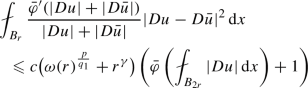and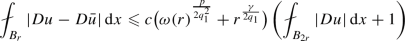for some $$c\geqslant 1$$ and $$\gamma \in (0,1)$$ depending only on n, p, q, L and $$\bar{L}$$.

### Proof

By monotonicity (3.3) and (Inc)$$_{p-1}$$ and (Dec)$${_{q_1}-1}$$ of $${\bar{\varphi }}'$$,

\begin{aligned}\begin{aligned} {\bar{F}}(\xi _1)-{\bar{F}}(\xi _2) -D_\xi {\bar{F}}(\xi _2) \cdot (\xi _1-\xi _2)&= \int _0^{1}( D_\xi {\bar{F}}(t\xi _1+(1-t)\xi _2) - D_\xi {\bar{F}}(\xi _2) )\cdot (\xi _1-\xi _2)\,\mathrm{d}t \\&\gtrsim \int _0^{1} t\frac{{\bar{\varphi }}'(|t\xi _1+(1-t)\xi _2|+|\xi _2|)}{|t\xi _1+(1-t)\xi _2| +|\xi _2|}|\xi _1-\xi _2|^2\,\mathrm{d}t \\&\gtrsim \int _{3/4}^{1} t\frac{{\bar{\varphi }}'(|t\xi _1+(1-t)\xi _2|+|\xi _2|)}{|\xi _1|+|\xi _2|}|\xi _1-\xi _2|^2\,\mathrm{d}t\\&\gtrsim \left( \int _{3/4}^{1} t\,\mathrm{d}t\right) \frac{{\bar{\varphi }}'(\frac{3}{4}|\xi _1|-\frac{1}{4}|\xi _2|+|\xi _2|)}{|\xi _1|+|\xi _2|}|\xi _1-\xi _2|^2 \\&\approx \frac{{\bar{\varphi }}'(|\xi _1|+|\xi _2|)}{|\xi _1|+|\xi _2|}|\xi _1-\xi _2|^2 \end{aligned} \end{aligned}

for every $$\xi _1,\xi _2\in {\mathbb {R}^n}$$. Using this and the facts that $$u-{\bar{u}}\in W^{1,\varphi }_0(B_r) \subset W^{1,{\bar{\varphi }}}_0(B_r)$$ from Lemma 6.1, we find that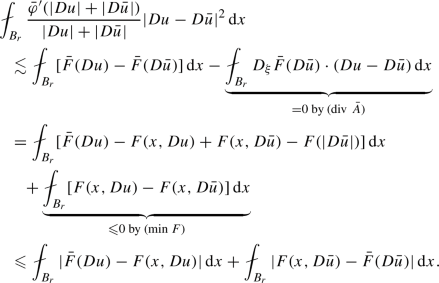We shall estimate only the second integral; the estimate for the first is analogous, we merely swap the roles of $$D{\bar{u}}$$ and Du. We split $$B_r$$ into

\begin{aligned} \begin{aligned} E_1{:=}B_r \cap \left\{ \varphi ^-_{B_r}(|D{\bar{u}}|)\leqslant \frac{|B_{r}|^{-1+\varepsilon }}{2^p}\right\} \text {and} E_2{:=}B_r \cap \left\{ \frac{|B_{r}|^{-1+\varepsilon }}{2^p }<\varphi ^-_{B_r}(|D{\bar{u}}|)\right\} . \end{aligned} \end{aligned}

In the set $$E_1$$, $$|D{\bar{u}}|\leqslant \frac{t_2}{2}$$. If also $$2t_1 \leqslant |D{\bar{u}}|$$, then $${\bar{F}}(\xi )=F(x_0,\xi )$$ and (6.6) imply that

\begin{aligned} |F(x,D{\bar{u}})- {\bar{F}}(D{\bar{u}})| = |F(x,D{\bar{u}})- F(x_0,D{\bar{u}})| \lesssim \omega (r) (\varphi ^-_{B_r}(|D{\bar{u}}|)+1). \end{aligned}

When $$|D{\bar{u}}|< 2t_1$$, we use $$F\approx \varphi$$, $${\bar{F}}\approx {\bar{\varphi }}$$ and that $$\varphi$$ and $${\bar{\varphi }}$$ satisfy (A0), (Inc)$$_{p}$$ and (Dec)$$_{q_1}$$, to conclude that $$|F(x,D{\bar{u}})- {\bar{F}}(D{\bar{u}})|\lesssim \omega (r)^\frac{p}{q_1}$$. Therefore, applying Lemma 6.1(1) &(2), we have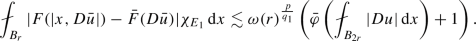In the set $$E_2$$, Proposition 5.1(3), $$1\lesssim |B_{2r}|^{1-\varepsilon }\varphi ^-_{B_r}(|D{\bar{u}}|)$$ and $$F\approx \varphi$$ imply that

\begin{aligned} |F(x,D{\bar{u}})- {\bar{F}}(D{\bar{u}})| \lesssim \varphi (x,|D{\bar{u}}|) \lesssim r^{\frac{n(1-\varepsilon )\sigma }{2}} \varphi (x,|D{\bar{u}}|)^{1+\frac{\sigma }{2}}. \end{aligned}

Integrating this inequality over $$E_2$$ and using the definition of $$\varepsilon$$ from (6.2), we find thatIn the same way as in the proof of Lemma 6.2, we derive from this that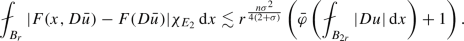Adding the estimates in $$E_1$$ and $$E_2$$, we obtain the inequality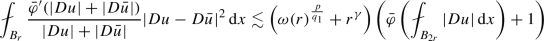with $$\gamma {:=}\frac{n\sigma ^2}{4(2+\sigma )}$$. This is the first claim of the lemma. The second claim follows from the first one by the same argument as in the proof of Lemma 6.2.

### Proof of Theorem 1.6

The proof is exactly same as that of Theorem 1.2, with Lemma 6.2 replaced by Lemma 6.3.

## 7 Examples

We present two known, important nonstandard growth problems, and show that they are special cases of Theorem 1.2 or 1.6. Various other examples of growth functions together with references about regularity results for related equations and minimizing problems can be found in .

The first example is the equation ($$\mathrm {div}A$$) with p(x)-growth, for which we refer to  (see also ). The second example is the functional ($$\min F$$) with the double phase condition, for which we refer to  (see also [8, 9]). For brevity, we consider one example in PDE form and the other as a minimizer, although obviously both problems could be considered in either form. In Example 7.1, p is a function whereas the lower growth exponent is denoted by $$p^-$$.

### Example 7.1

(Variable exponent growth) Let $$p:\Omega \rightarrow [p^-,p^+]$$ for some $$1<p^-\leqslant p^+$$, and let $$\omega _p$$ be the modulus of continuity of p and satisfy

\begin{aligned} \lim _{r\rightarrow 0} \omega _p(r)\ln \left( \tfrac{1}{r}\right) =0. \end{aligned}

Suppose $$A:\Omega \times {\mathbb {R}^n} \rightarrow {\mathbb {R}^n}$$ satisfies that $$A(x,\cdot )\in C^1({\mathbb {R}^n} \setminus \{0\},\mathbb {R}^n)$$ and

\begin{aligned} {\left\{ \begin{array}{ll} |A(x,\xi )|+ |\xi | |D_\xi A(x,\xi )| \leqslant L |\xi |^{p(x)-1},\\ |\xi |^{p(x)-2} |\tilde{\xi }|^2 \leqslant L D_\xi A(x,\xi ) \tilde{\xi }\cdot \tilde{\xi }, \\ |A(x,\xi )-A(y,\xi )| \leqslant L \omega _p(|x-y|)(|\xi |^{p(x)-1}+|\xi |^{p(y)-1})\big (1+\big |\ln |\xi |\,\big |\big ). \end{array}\right. } \end{aligned}

For instance, $$A(x,\xi )=|\xi |^{p(x)-2}\xi$$ satisfies these conditions.

We will show that A satisfies the assumptions of Theorem 1.2. Since the first two conditions above imply

\begin{aligned} |\xi |^{p(x)-2} \approx |D_\xi A(x,\xi )| \approx |\xi |^{-1}|A(x,\xi )| \quad \text {and} \quad |D_\xi A(x,\xi )|\lesssim D_\xi A(x,\xi ) e \cdot e, \end{aligned}

Assumption 1.1 is satisfied. We next derive (wVA1) for $$A^{(-1)}$$. Let $$r\in (0,1)$$ and $$x,y\in B_r$$. The third condition and $$|\xi |^{p(x)-2} \approx |\xi |^{-1}|A(x,\xi )|$$ imply that

\begin{aligned} |\xi |\,|A(x,\xi )-A(y,\xi )| \lesssim \omega _p(r)\big (1+\big |\ln |\xi |\big |\big ) |\xi |\,|A(y,\xi )| \end{aligned}

for all $$\xi \in {\mathbb {R}^n}$$. Moreover, if $$1\leqslant |\xi |\,|A(y,\xi )|\leqslant |B_r|^{-1}$$, then $$|\xi |^{p^-}\leqslant C r^{-n}$$ for some $$C\geqslant 1$$ so that

\begin{aligned} 1+\ln |\xi | \leqslant 1+ \tfrac{1}{p^-}\ln C + \tfrac{n}{p^-}\ln \left( \tfrac{1}{r}\right) \lesssim \ln \left( \tfrac{1}{r}\right) . \end{aligned}

On the other hand, if $$0\leqslant |\xi | |A(y,\xi )|\leqslant 1$$, then $$|\xi | |A(y,\xi )| \lesssim |\xi |^{p^-}$$ hence

\begin{aligned} \big (1+\big |\ln |\xi |\big |\big )\, |\xi |\,|A(y,\xi )| \lesssim \big (1+\big |\ln |\xi |\big |\big )\, |\xi |^{p^-} \lesssim 1. \end{aligned}

Therefore we have

\begin{aligned}&|\xi |\,|A(x,\xi )-A(y,\xi )| \lesssim \omega _p(r)\ln \left( \tfrac{1}{r}\right) (|\xi |\,|A(y,\xi )|+1), \\&|\xi |\,|A(y,\xi )|\in (0,|B_r|^{-1}]. \end{aligned}

This implies $$A^{(-1)}$$ satisfies (wVA1) with $$\omega _\varepsilon (r)= \omega _p(r)\ln \left( \tfrac{1}{r}\right)$$ for all $$\varepsilon \in (0,1]$$.

We note that if $$\omega _p(r)\lesssim r^\beta$$ for some $$\beta >0$$, then $$\omega _\varepsilon (r)\lesssim r^{\beta _\varepsilon }$$ for any $$\beta _\varepsilon \in (0,\beta )$$.

### Example 7.2

(Double phase growth) Let $$H(x,t)=t^p+a(x)t^q$$ with $$a:\Omega \rightarrow [0,a^+]$$ satisfy that for some $$\beta \in (0,1]$$,

\begin{aligned} 1\leqslant \frac{q}{p}\leqslant 1+\frac{\beta }{n} \quad \text {and}\quad |a(x)-a(y)|\leqslant L|x-y|^\beta . \end{aligned}

Suppose $$F:\Omega \times {\mathbb {R}^n} \rightarrow \mathbb {R}$$ satisfies $$F(x,\cdot )\in C^1({\mathbb {R}^n} )\cap C^2({\mathbb {R}^n} \setminus \{0\})$$,

\begin{aligned} {\left\{ \begin{array}{ll} |\xi | \, |D_\xi F(x,\xi )| + |\xi |^2 \, |D_\xi ^2 F(x,\xi )| \leqslant L H(x,|\xi |), \\ |\xi |^{-2}H(x,|\xi |) |\tilde{\xi }|^2 \leqslant L D_\xi ^2F(x,\xi ) \tilde{\xi }\cdot \tilde{\xi }, \\ |F(x,\xi )-F(y,\xi )| \leqslant L \tilde{\omega }(|x-y|)(H(x,|\xi |)+H(y,|\xi |))+ L |a(x)-a(y)|\,|\xi |^q. \end{array}\right. } \end{aligned}

For instance, $$F(x,\xi )=\gamma (x) H(x,\xi _1^4+\cdots +\xi _n^4)$$ with $$\tilde{\omega }$$ the modulus of continuity of $$\gamma$$ satisfies these conditions if $$0<\gamma ^- \leqslant \gamma \leqslant \gamma ^+$$.

We will show that F satisfies the assumptions of Theorem 1.6. Assumption 1.5 is obvious from the first two conditions above. We next show (wVA1). Let $$r\in (0,1)$$ and $$x,y\in B_r$$. The first condition implies that

\begin{aligned} |F(x,\xi )-F(y,\xi )| \lesssim \tilde{\omega }(2r)(|H(x,\xi )|+|H(y,\xi )|)+ r^\beta |\xi |^q \end{aligned}

for all $$\xi \in {\mathbb {R}^n}$$. From the first and second conditions, we conclude that $$|H(x,\xi )| \lesssim F(x,\xi )$$ and $$|H(y,\xi )| \lesssim F(y,\xi )$$. If $$F(y,\xi )\leqslant |B_r|^{-1+\varepsilon }$$, then $$|\xi |^p\lesssim r^{-(1-\varepsilon )n}$$. Since $$\beta \geqslant \frac{(q-p)n}{p}$$, we have

\begin{aligned} r^\beta |\xi |^{q}\leqslant r^\beta |\xi |^{q-p}H(y,|\xi |) \lesssim r^{\frac{\varepsilon (q-p)n}{p}} F(y,|\xi |) \end{aligned}

Therefore we have

\begin{aligned} |F(x,\xi )-F(y,\xi )| \lesssim \left( \tilde{\omega }(2r)+r^{\frac{\varepsilon (q-p)n}{p}}\right) (|F(x,\xi )| + |F(y,\xi )|). \end{aligned}

From this estimate we obtain $$|F(x,\xi )| \lesssim |F(y,\xi )|$$, and this we have (wVA1) with $$\omega _\varepsilon (r)= \tilde{\omega }(2r)+r^{\frac{\varepsilon (q-p)n}{p}}$$.

We note that if $$\tilde{\omega }(r) \lesssim r^\beta$$ for some $$\beta >0$$, then $$\omega _\varepsilon (r)\lesssim r^{\min \{\beta , \frac{\varepsilon (q-p)n}{p}\}}$$.